# R S Aggarwal Solutions for Class 11 Maths Chapter 30 Statistics

R S Aggarwal solutions Class 11 Chapter 30 consists of questions and answers related to Statistics. This chapter is very helpful, not only for the purpose of final exams but for the preparation of competitive exams as well. All solutions to questions in R S Aggarwal Class 11 are given in a detailed and step by step format to help the students understand the concepts. In this chapter, students will learn to find the mean, median, standard deviation, variance using different formulas and many more. Click on the link below to download all the solutions.

R S Aggarwal Chapter 30 – Statistics, contains the answers to all the questions listed in this chapter. Let us have a look at some of the topics covered here:

• Statistics Introduction
• Mean Deviation
• Mean deviation for discrete frequency distribution
• Variance and standard deviation
• Coefficient of variance

## Download PDF of R S Aggarwal Solutions for Class 11 Maths Chapter 30 Statistics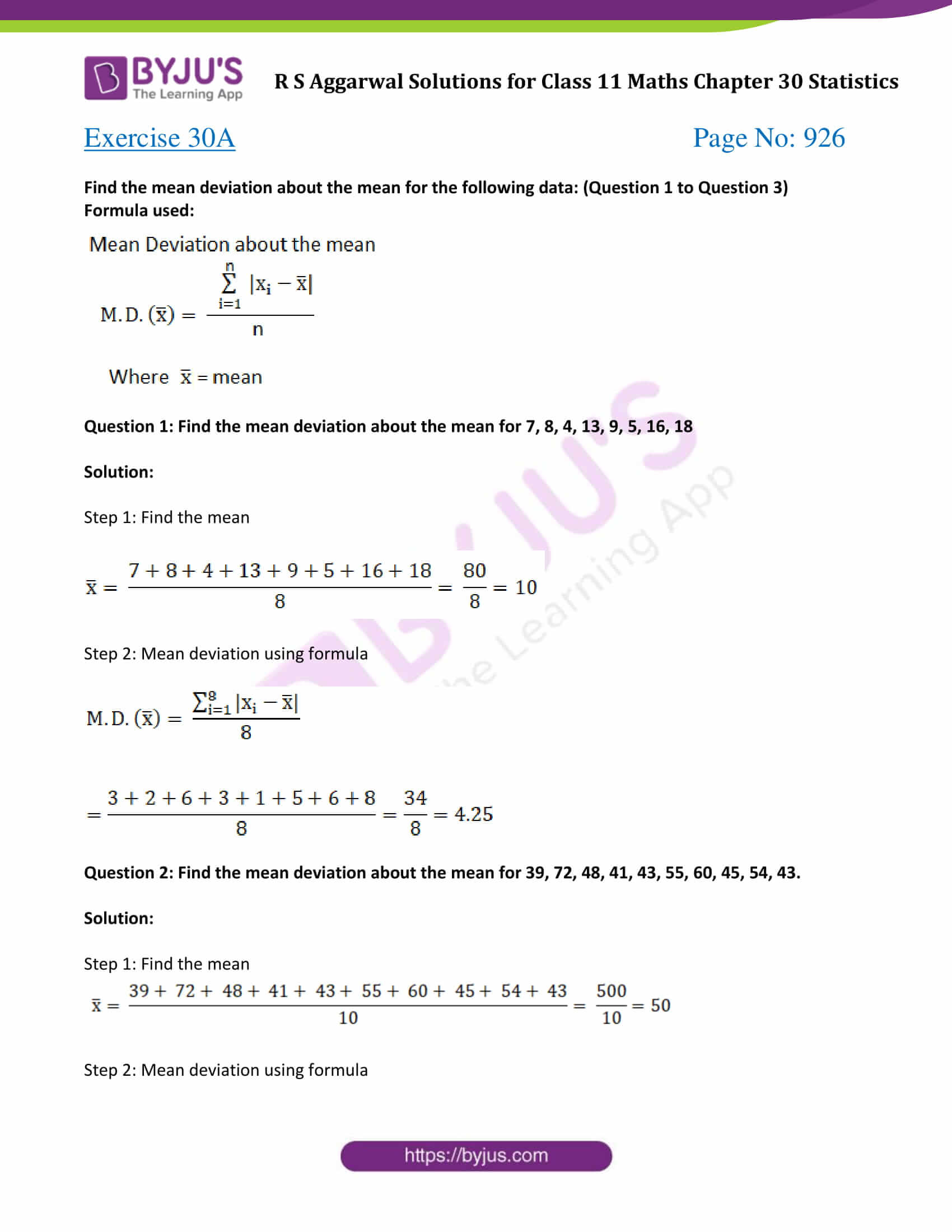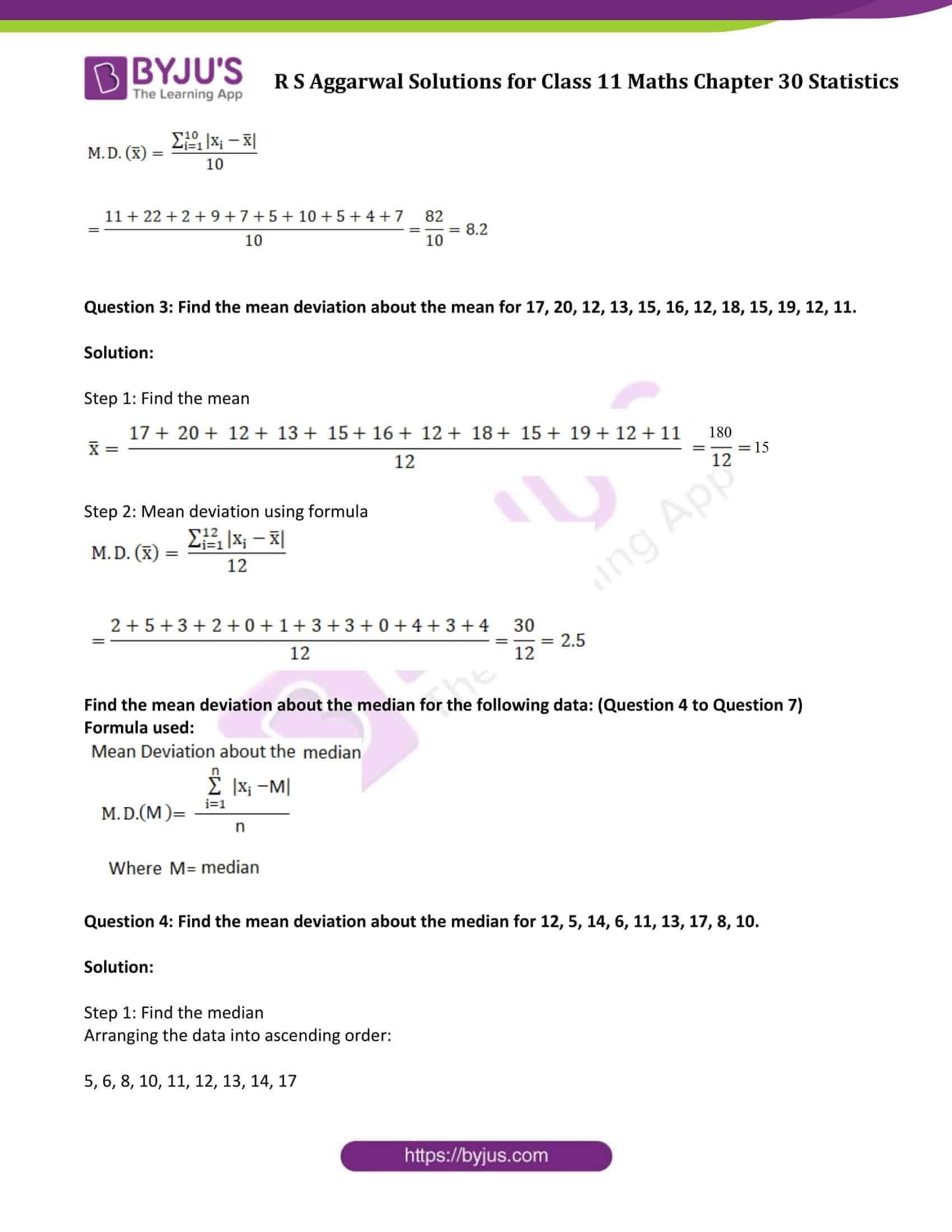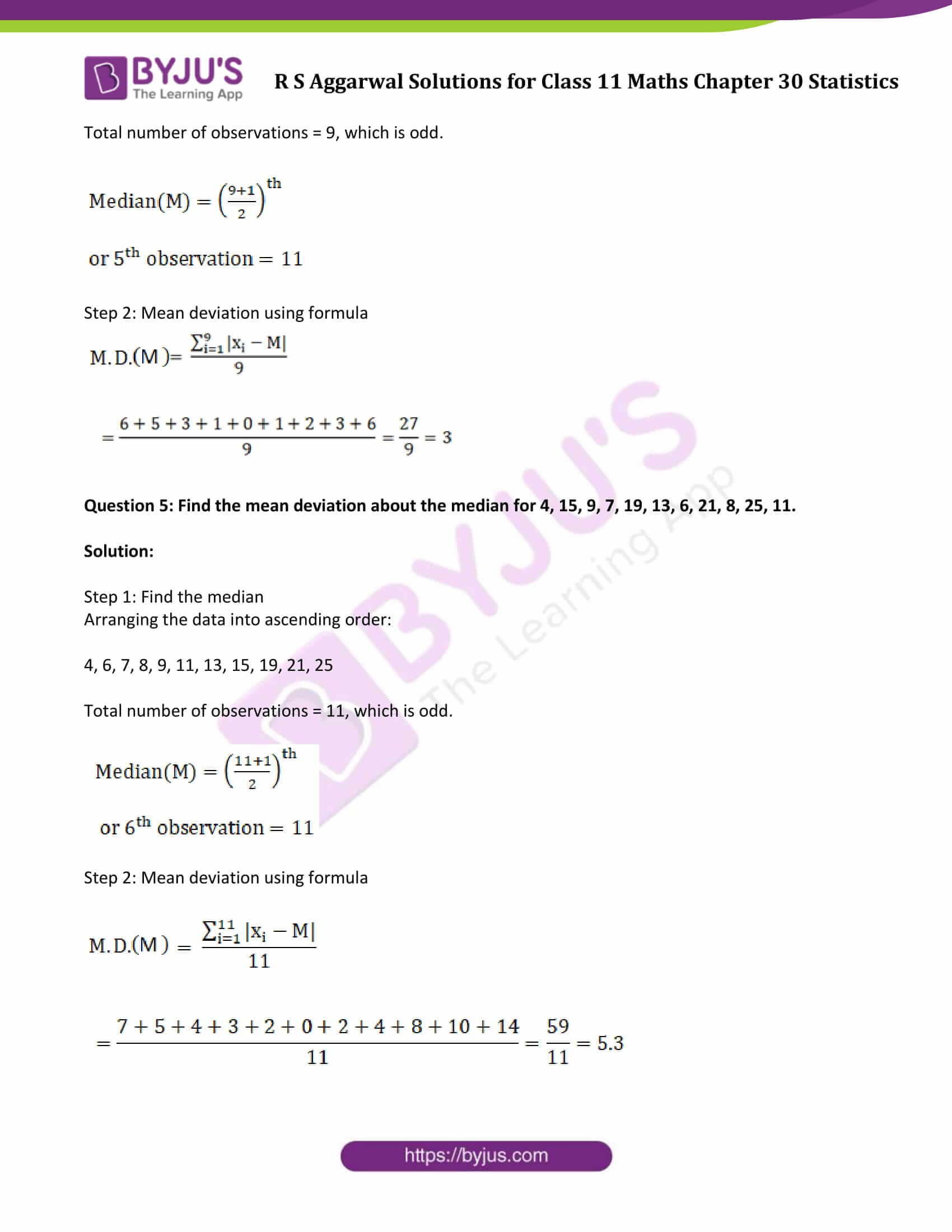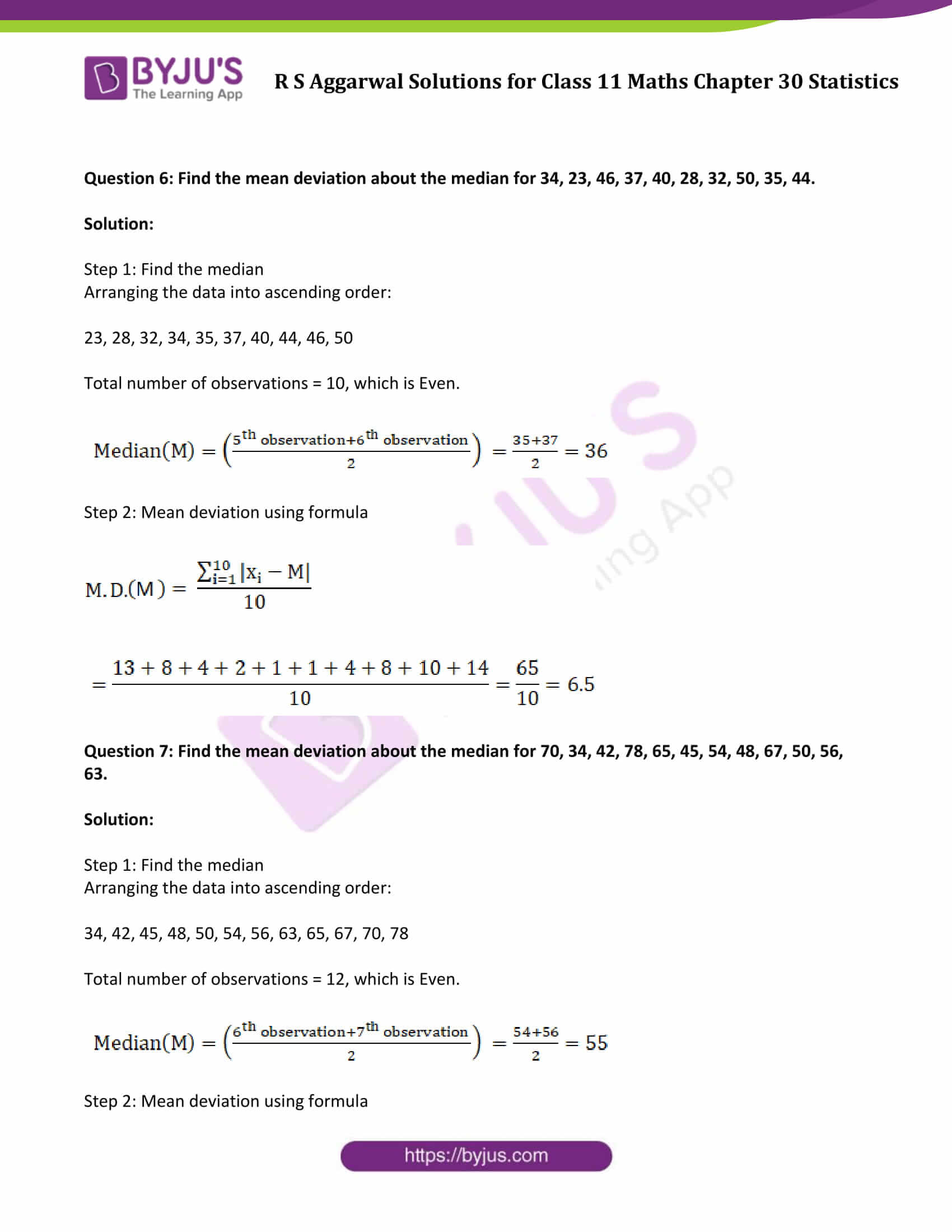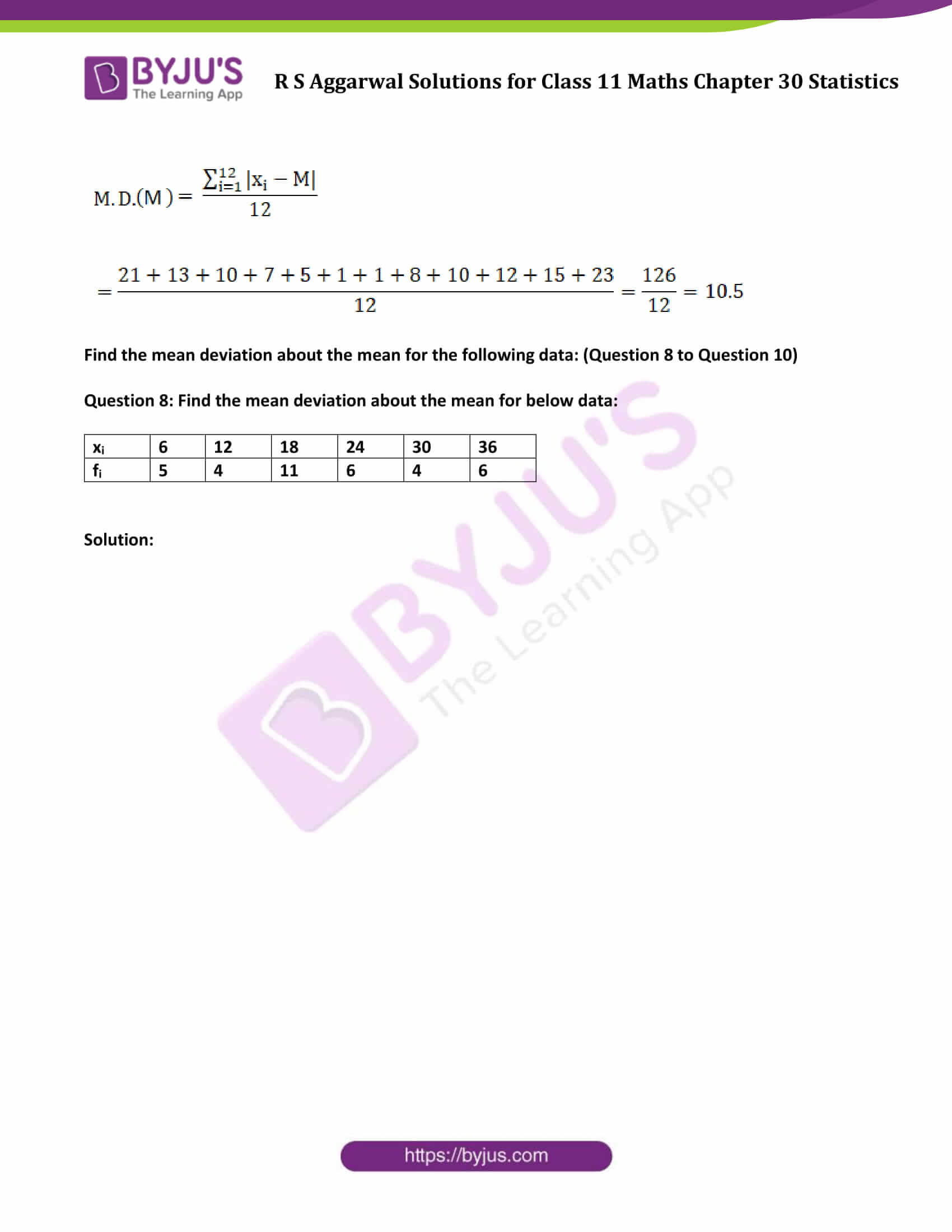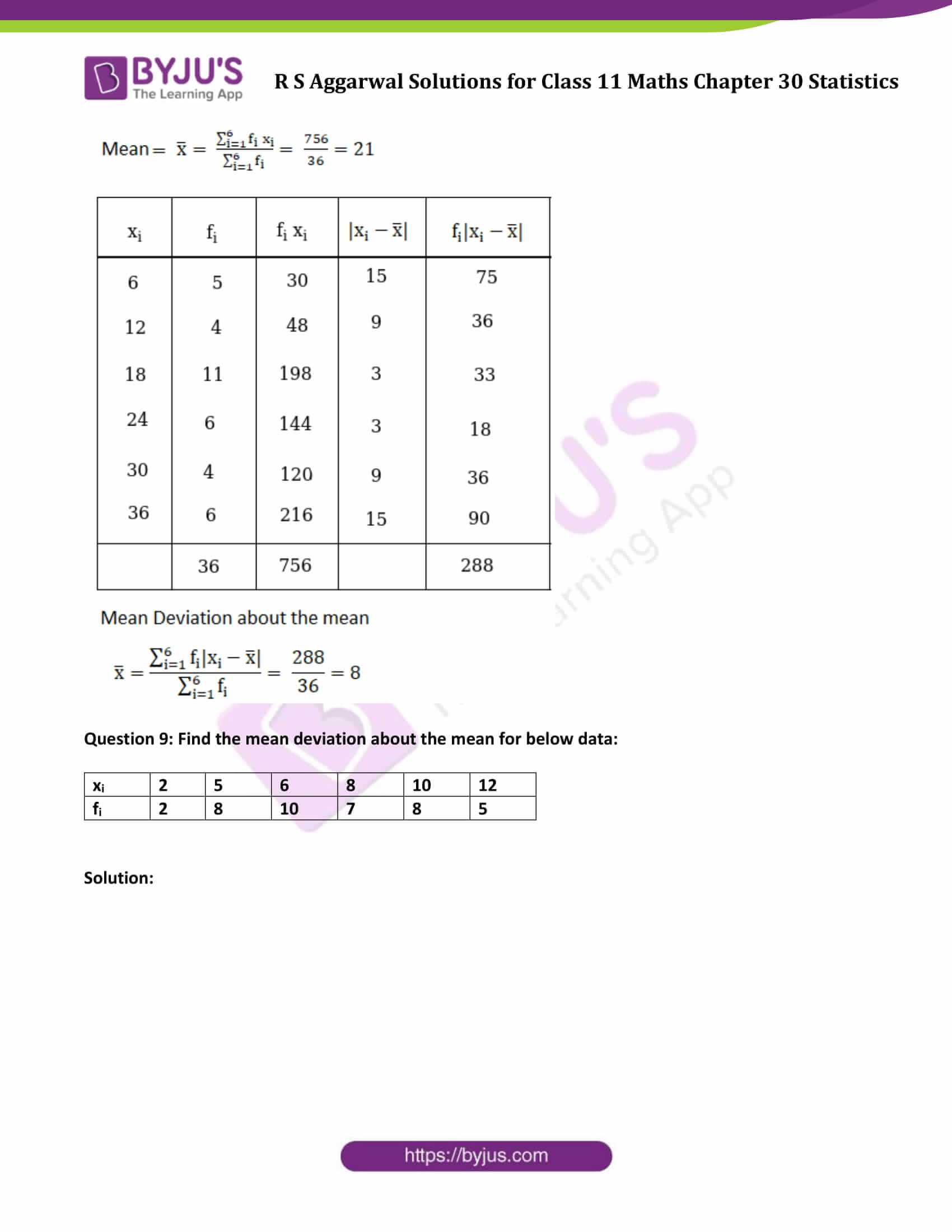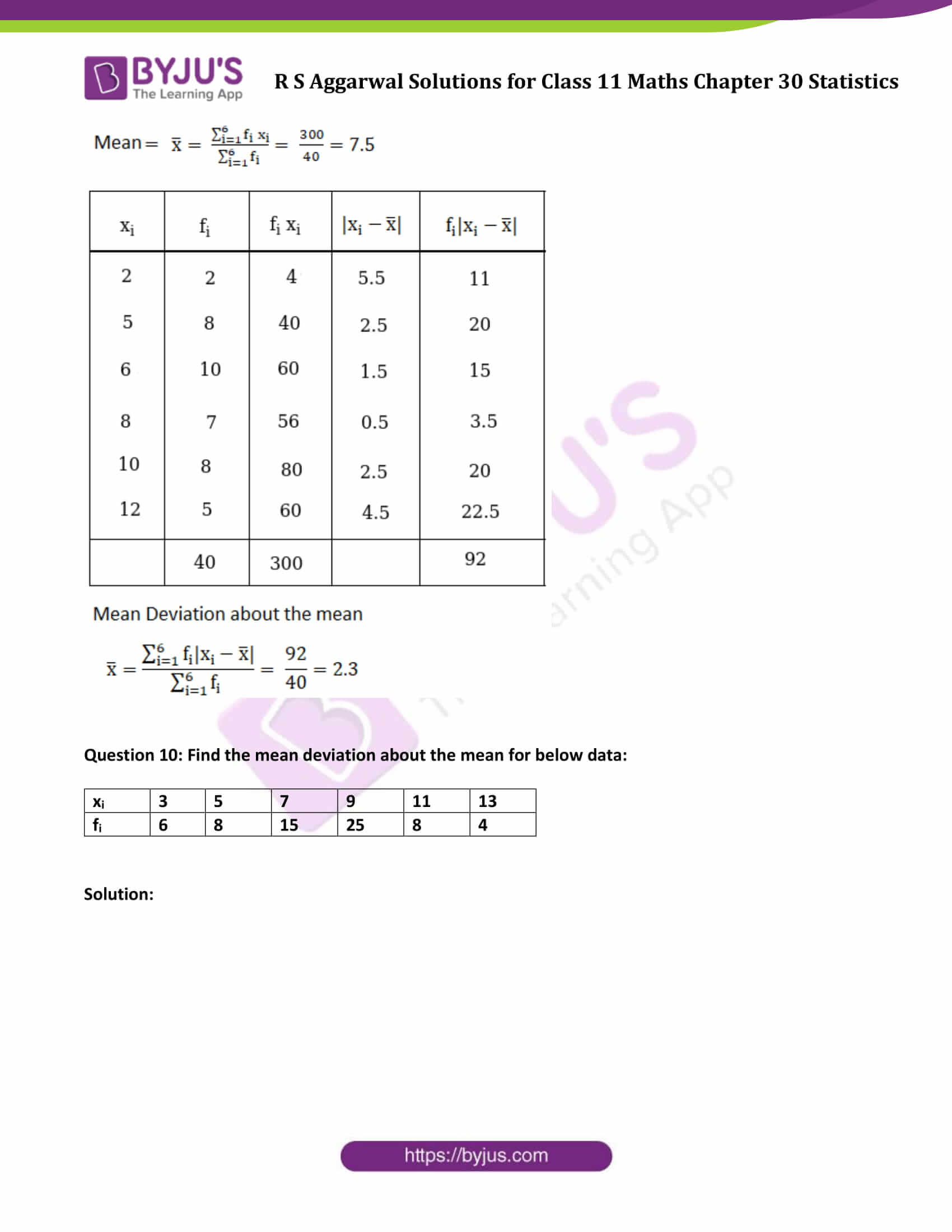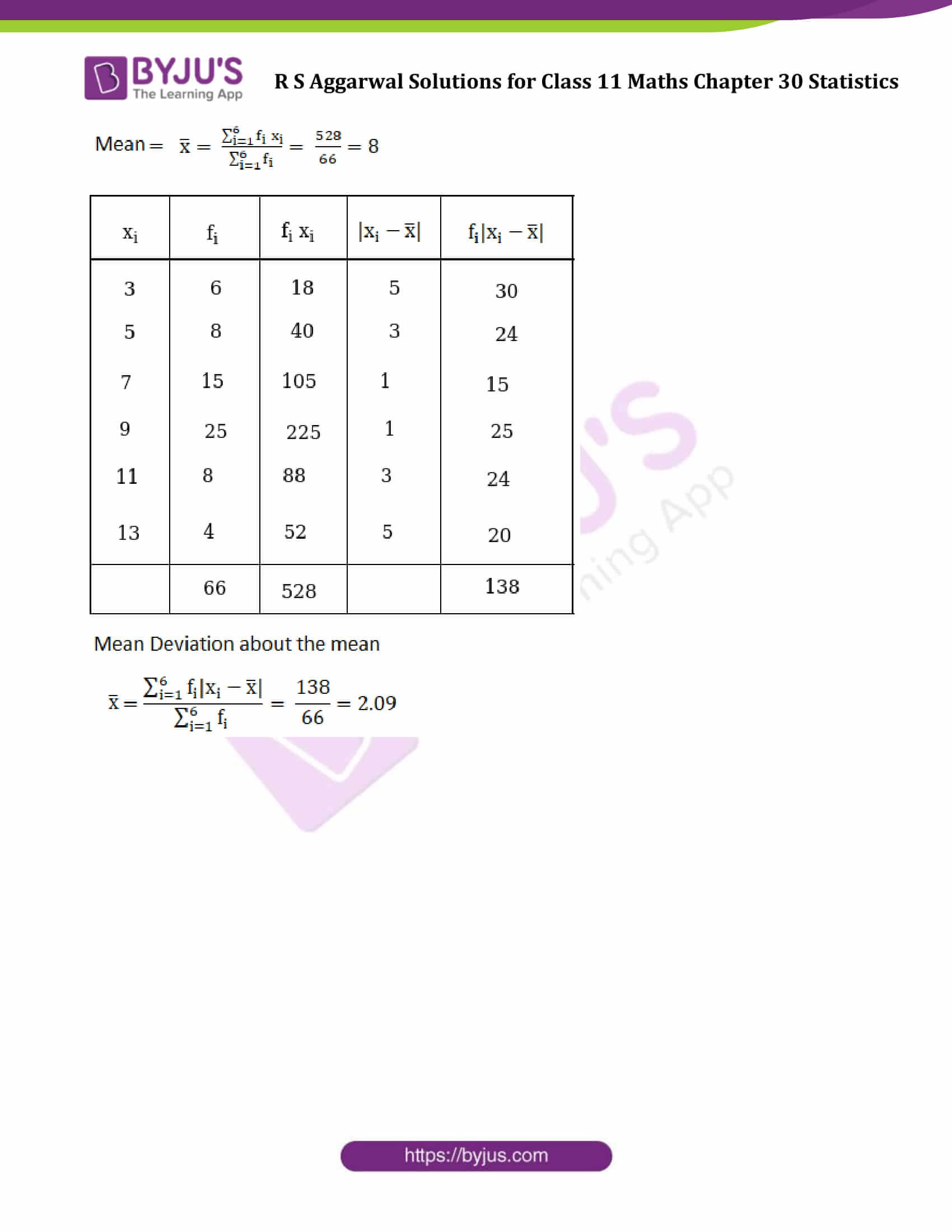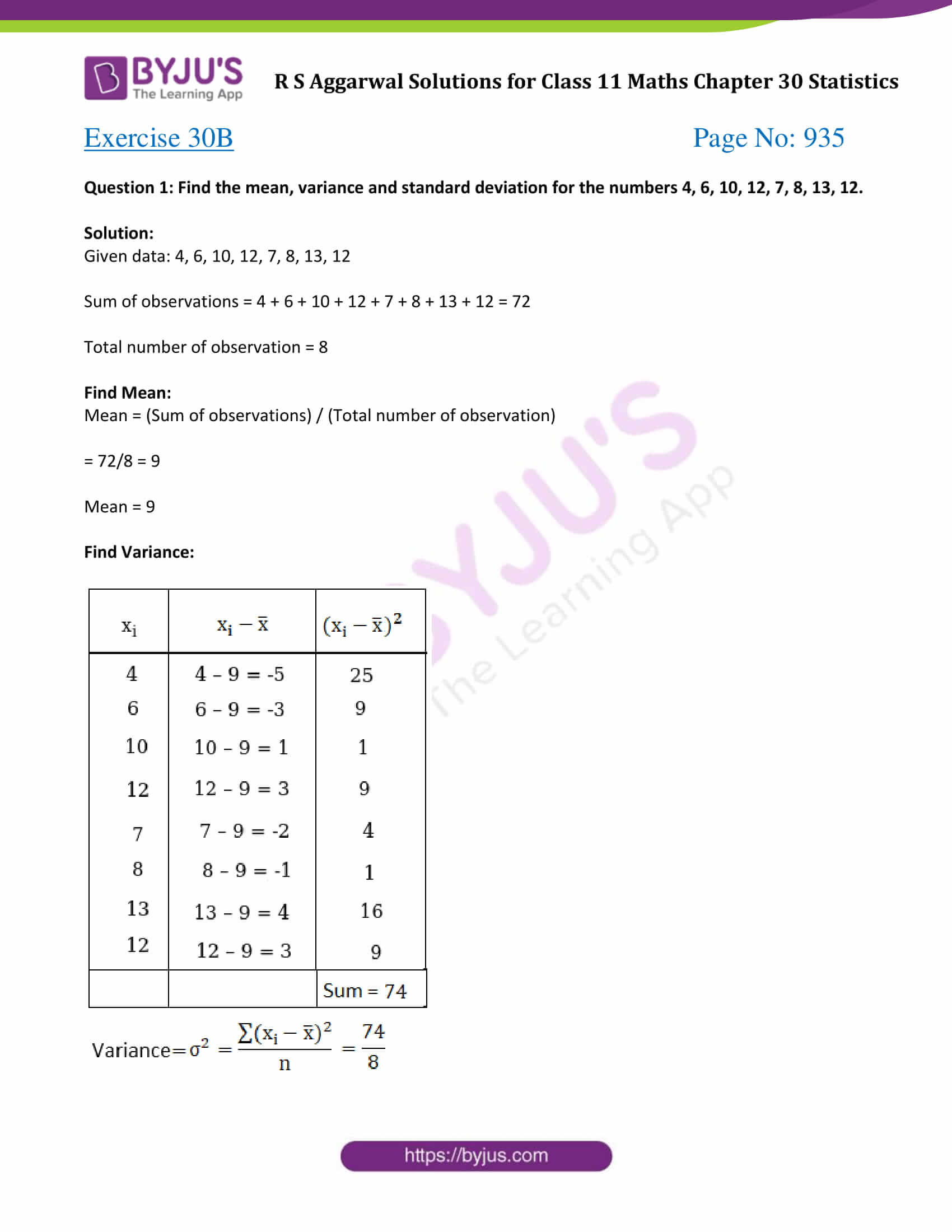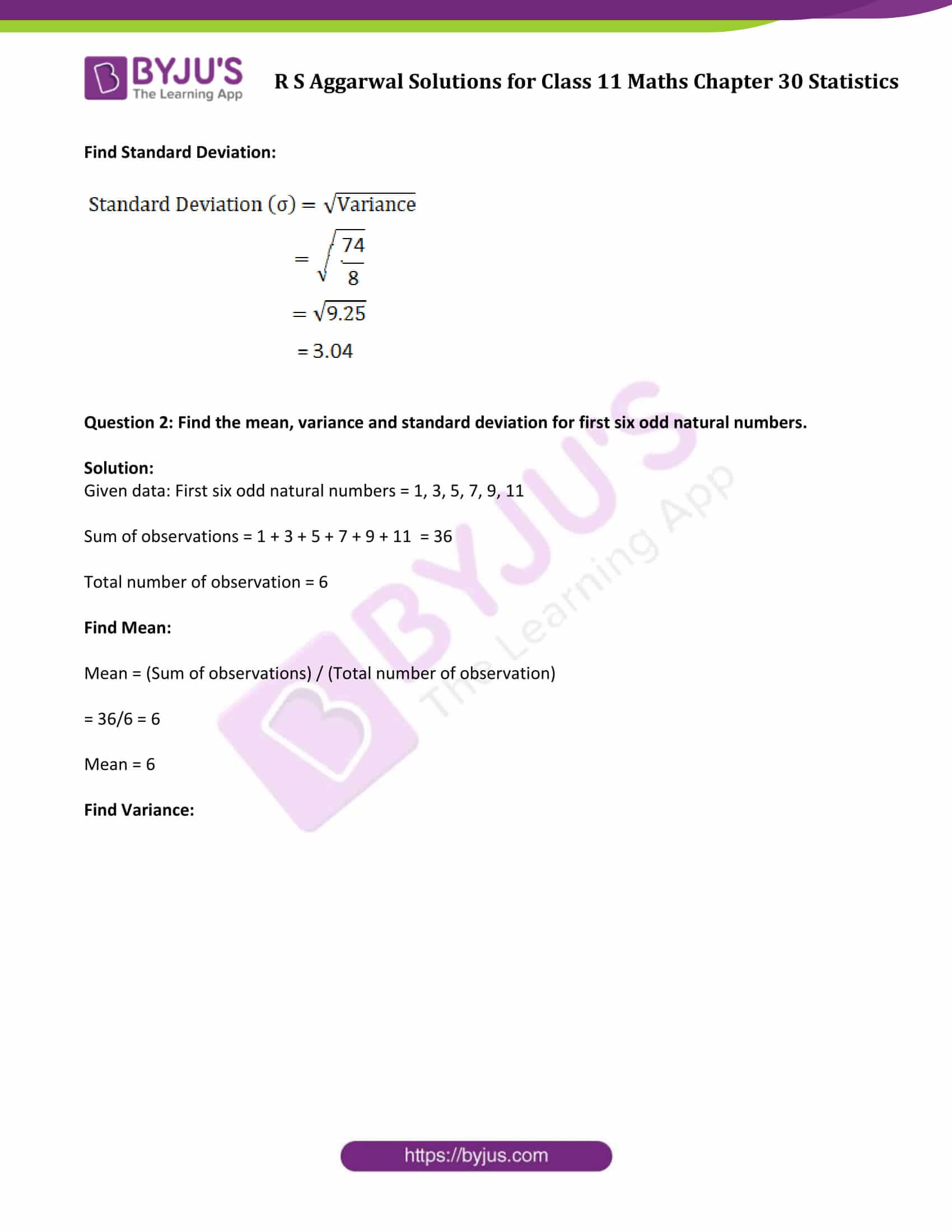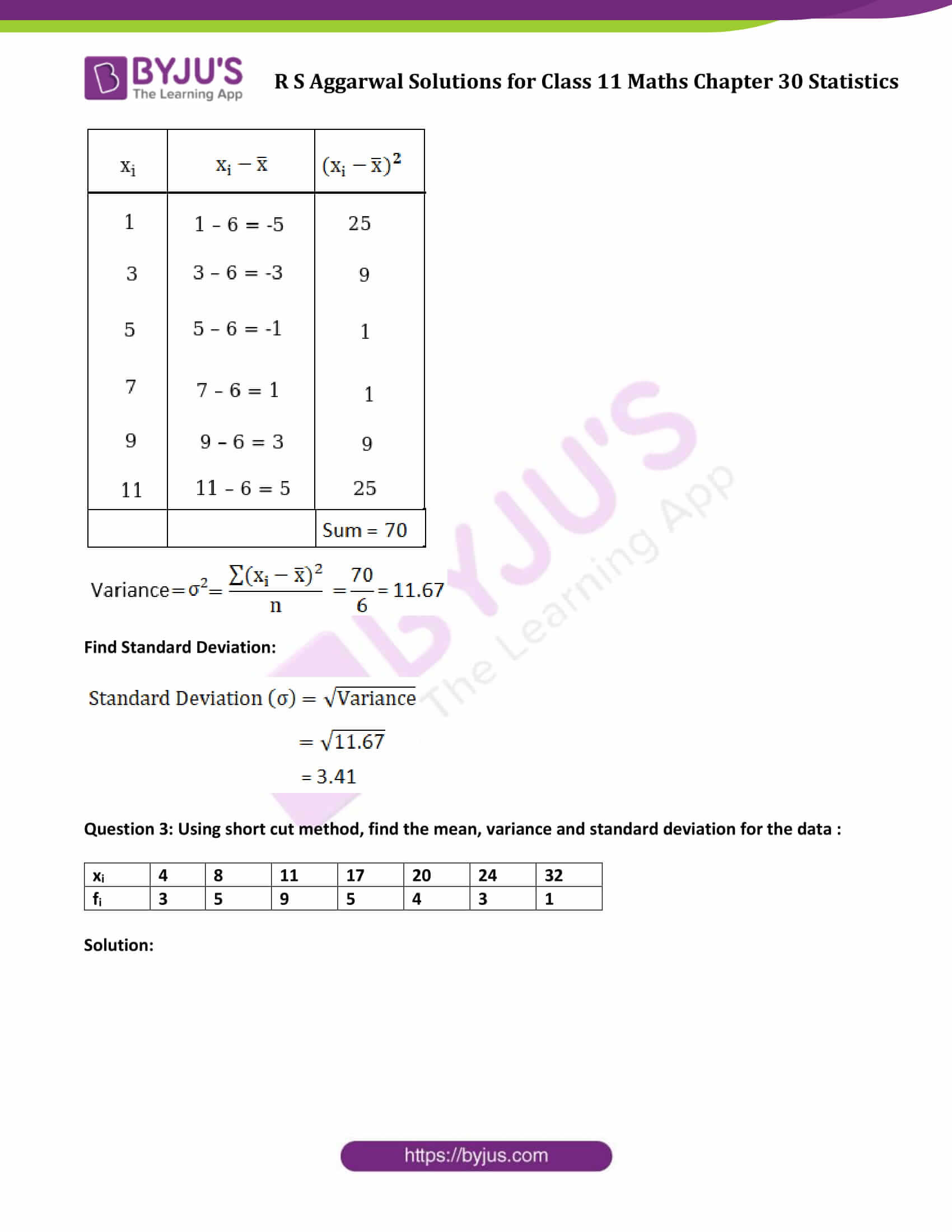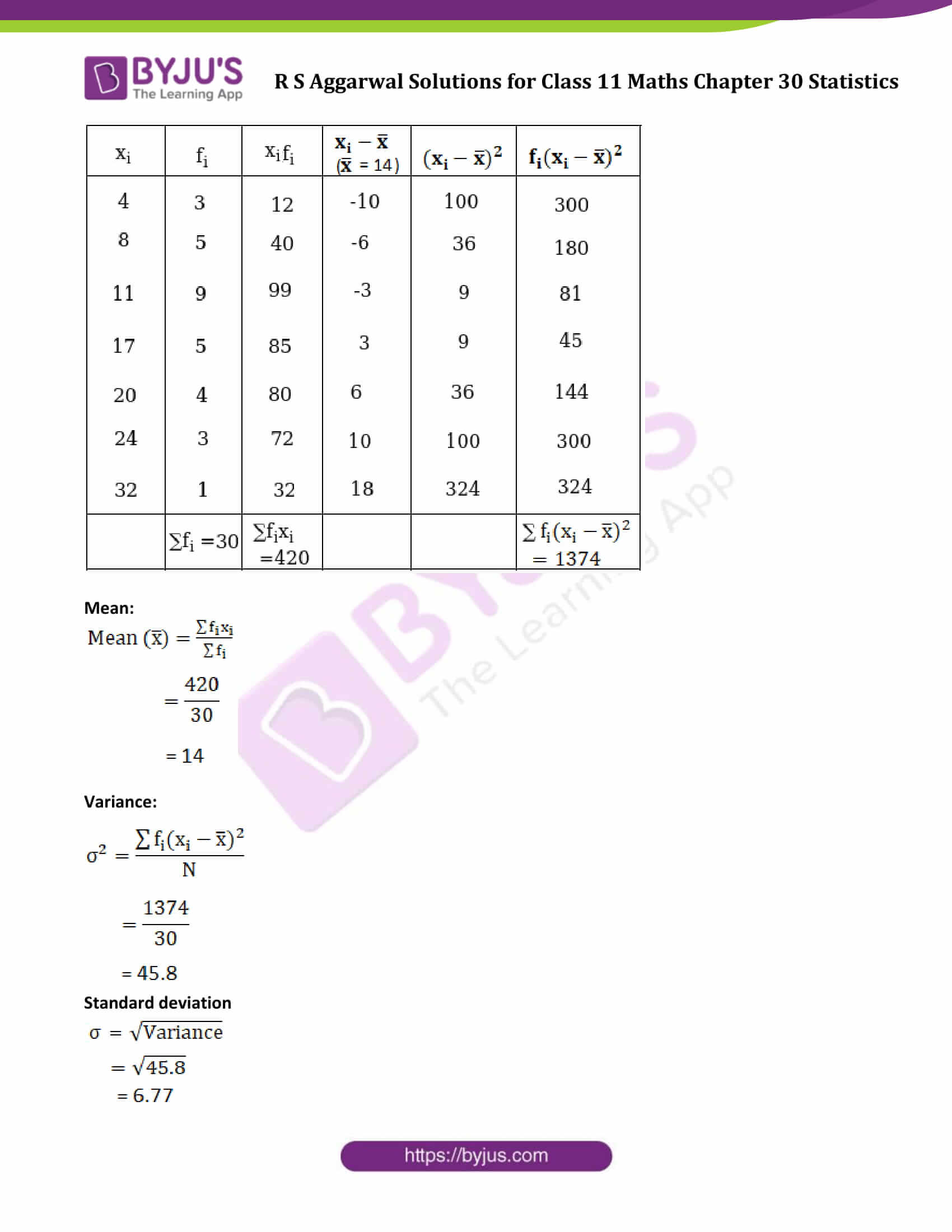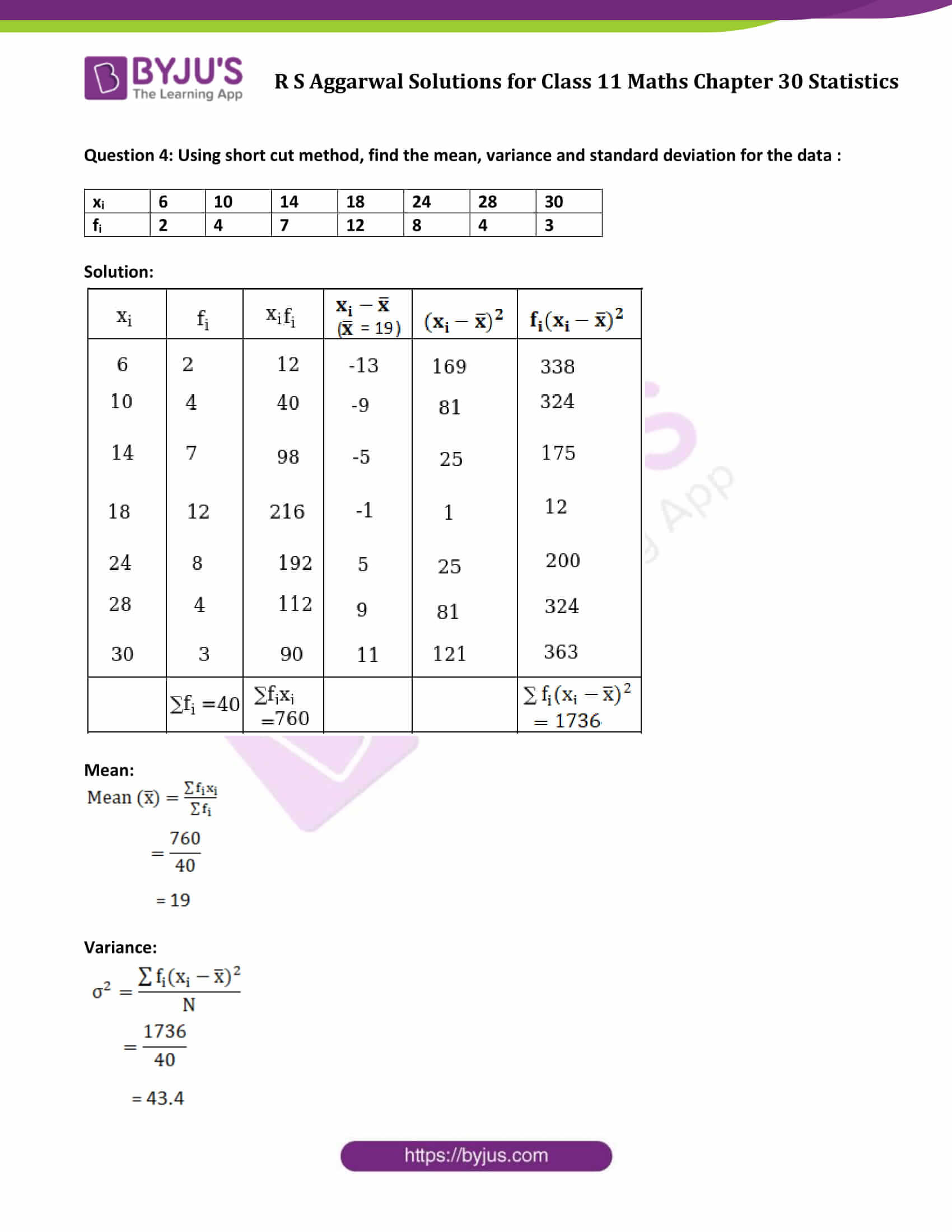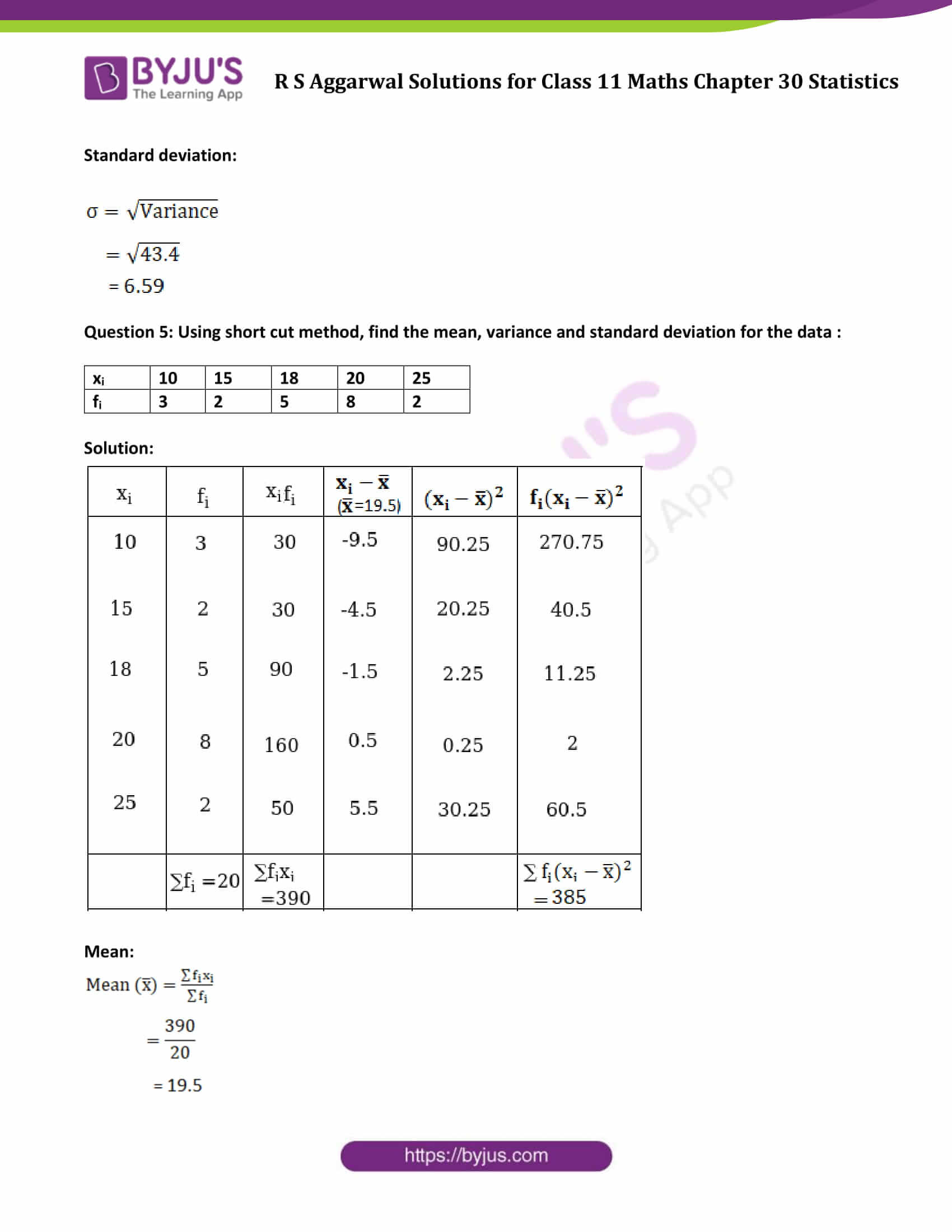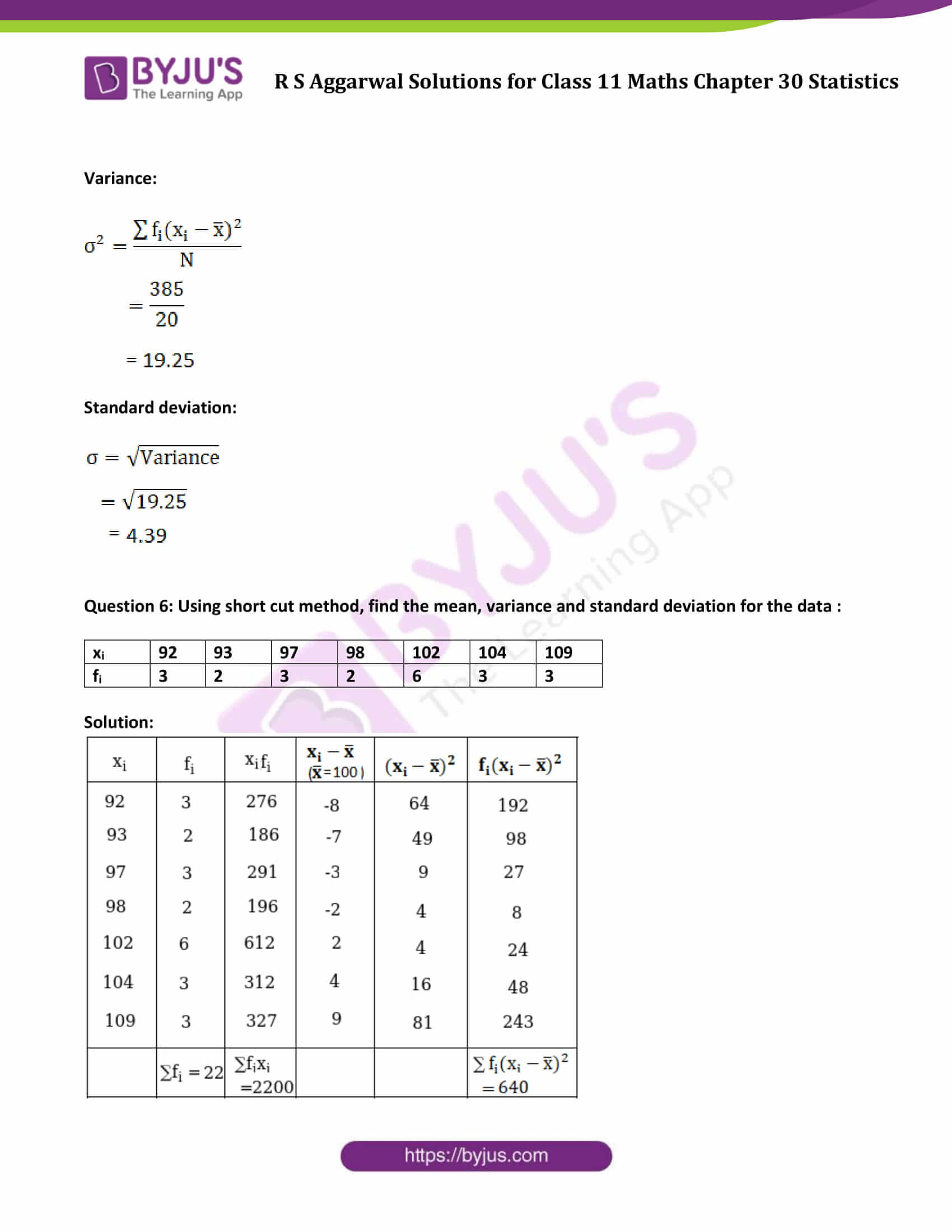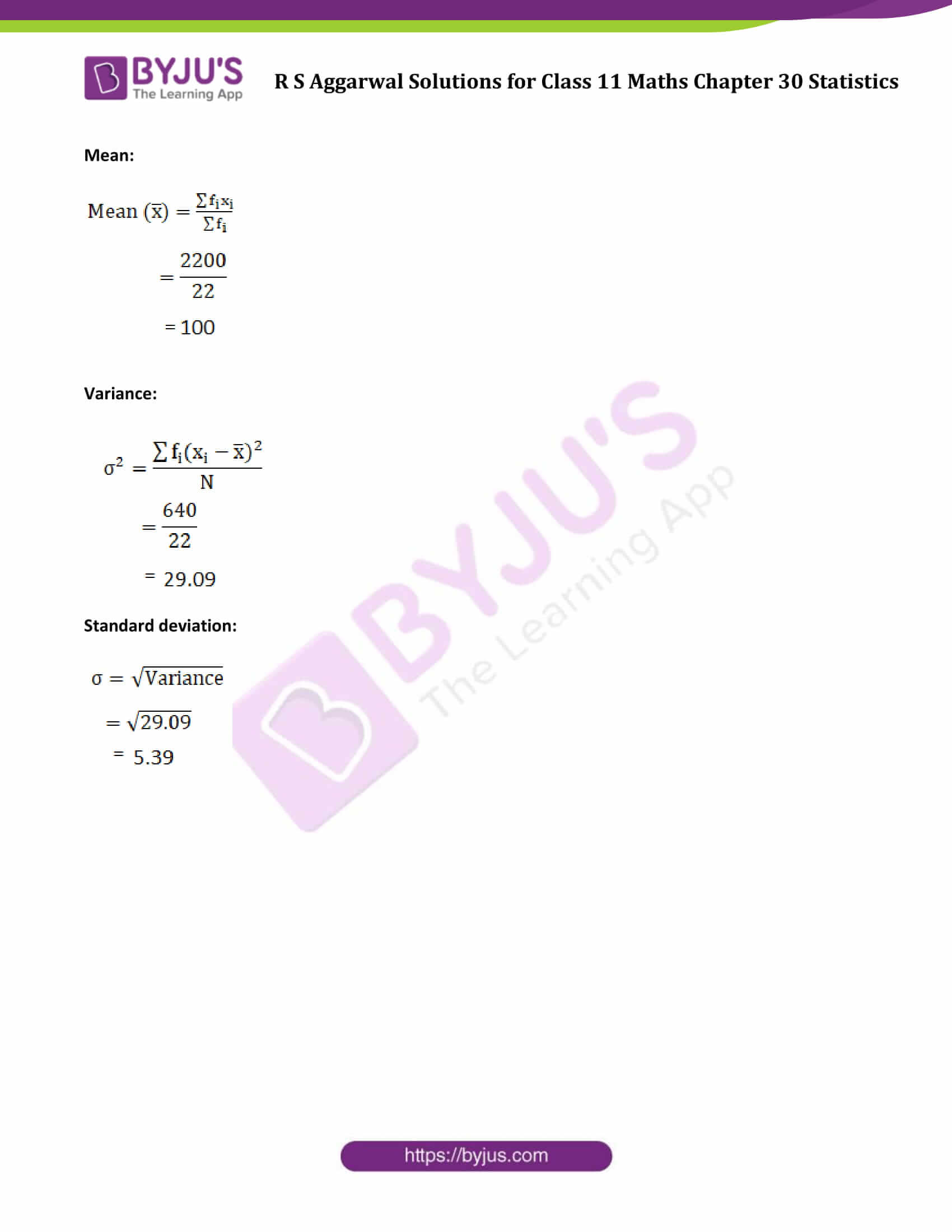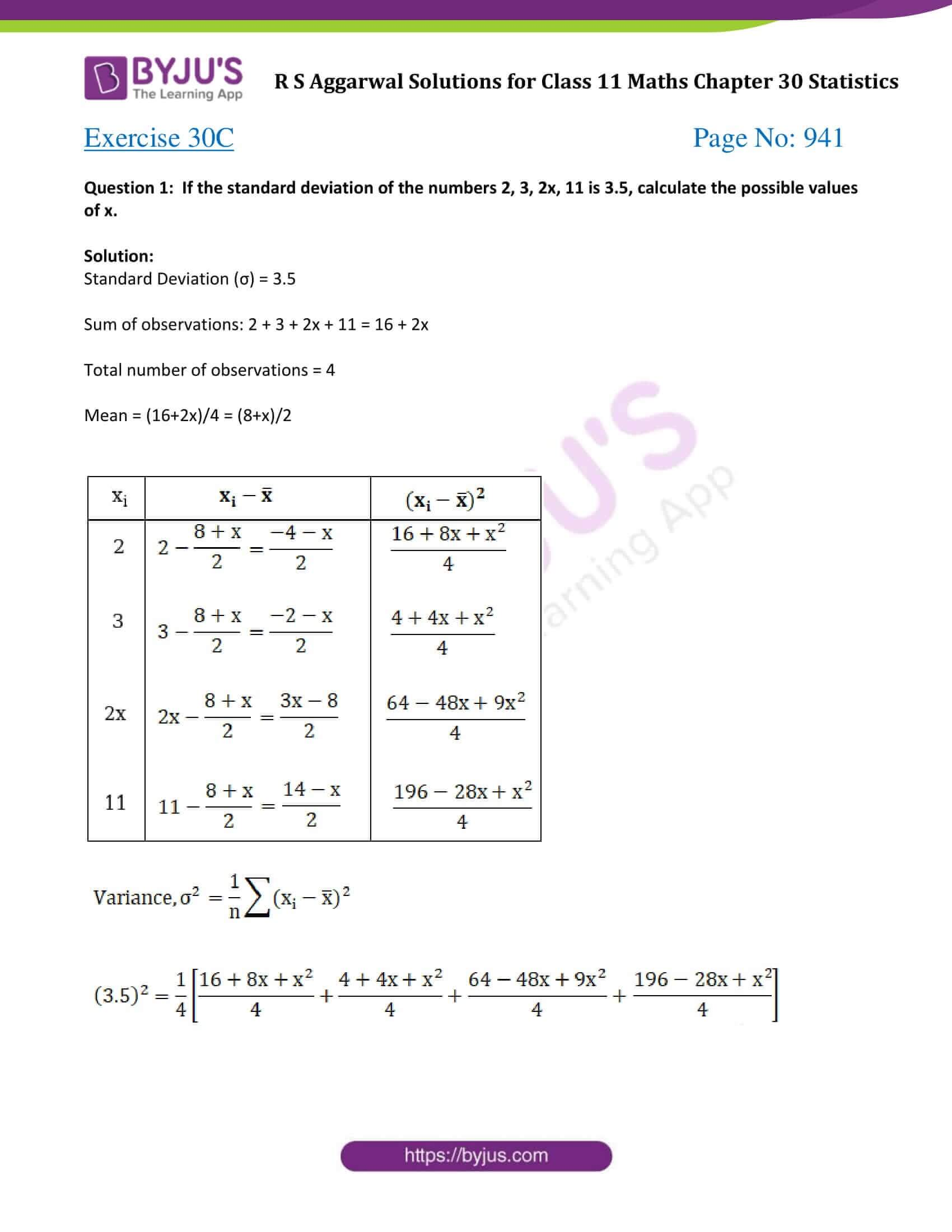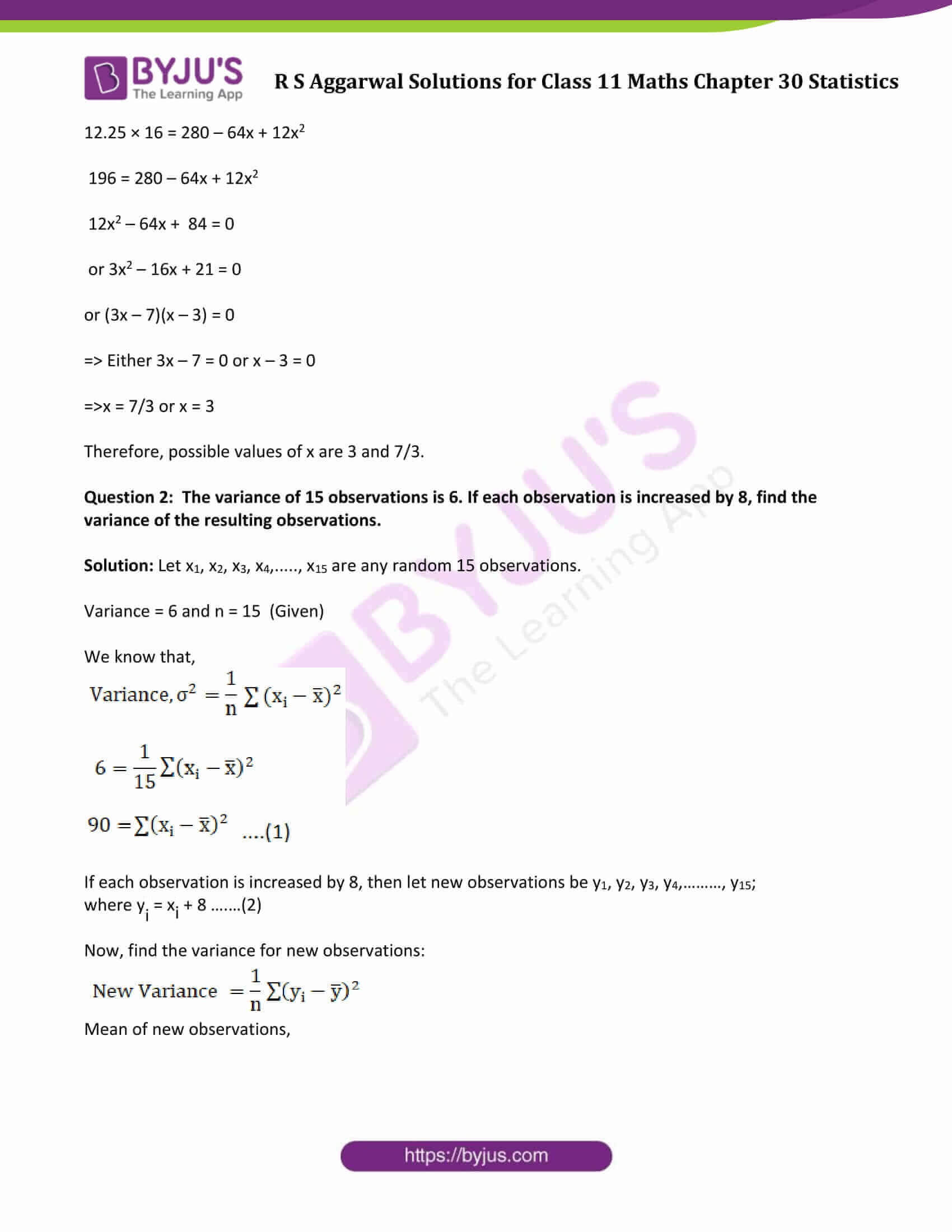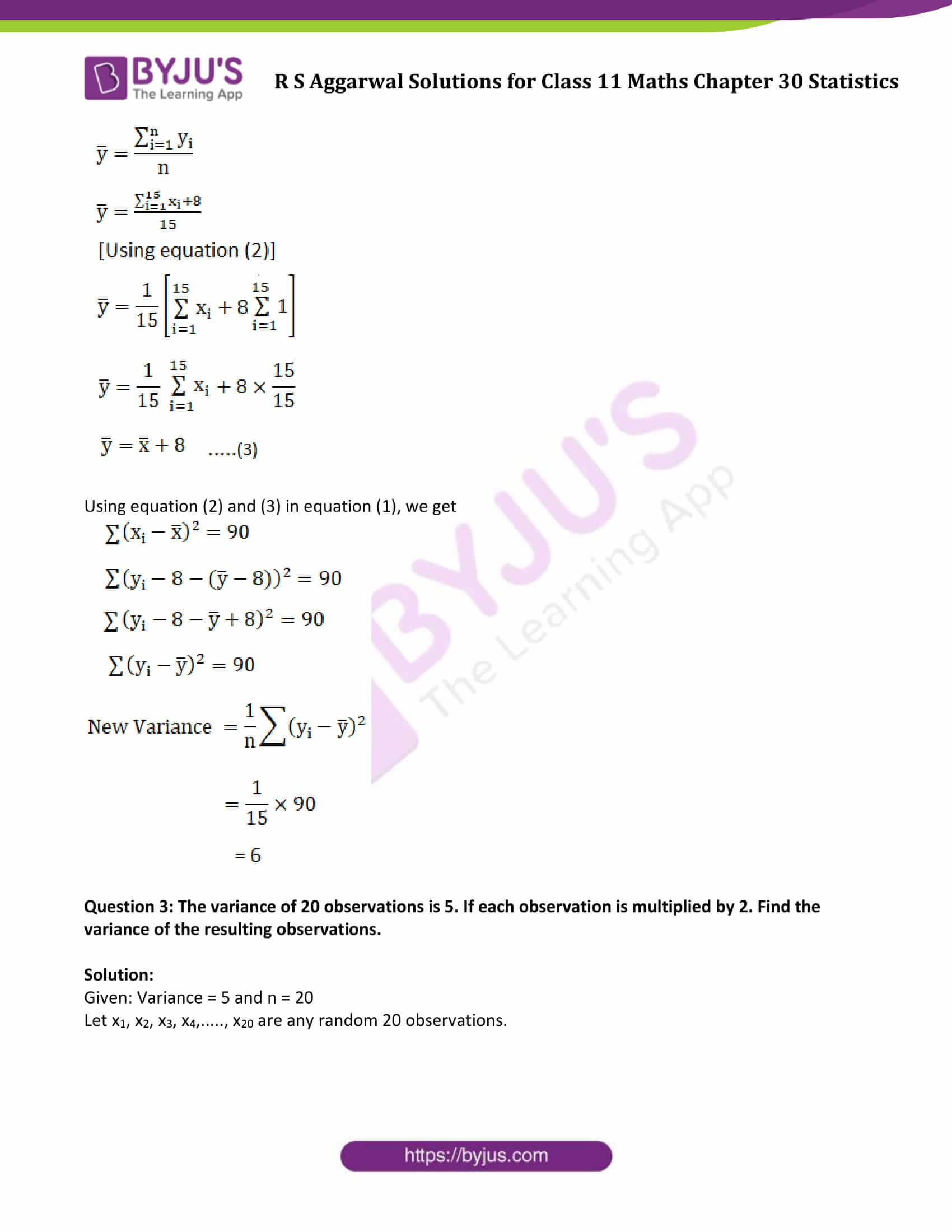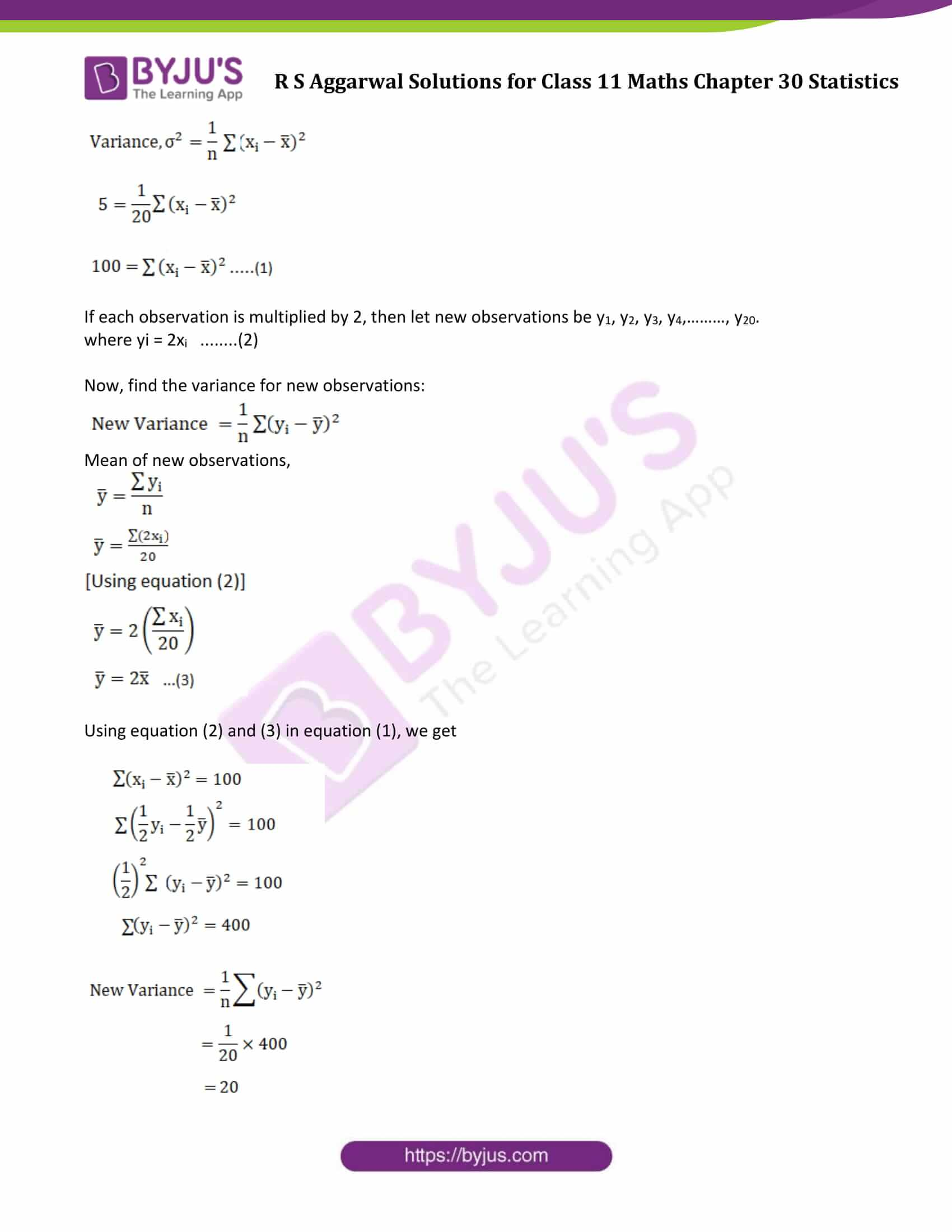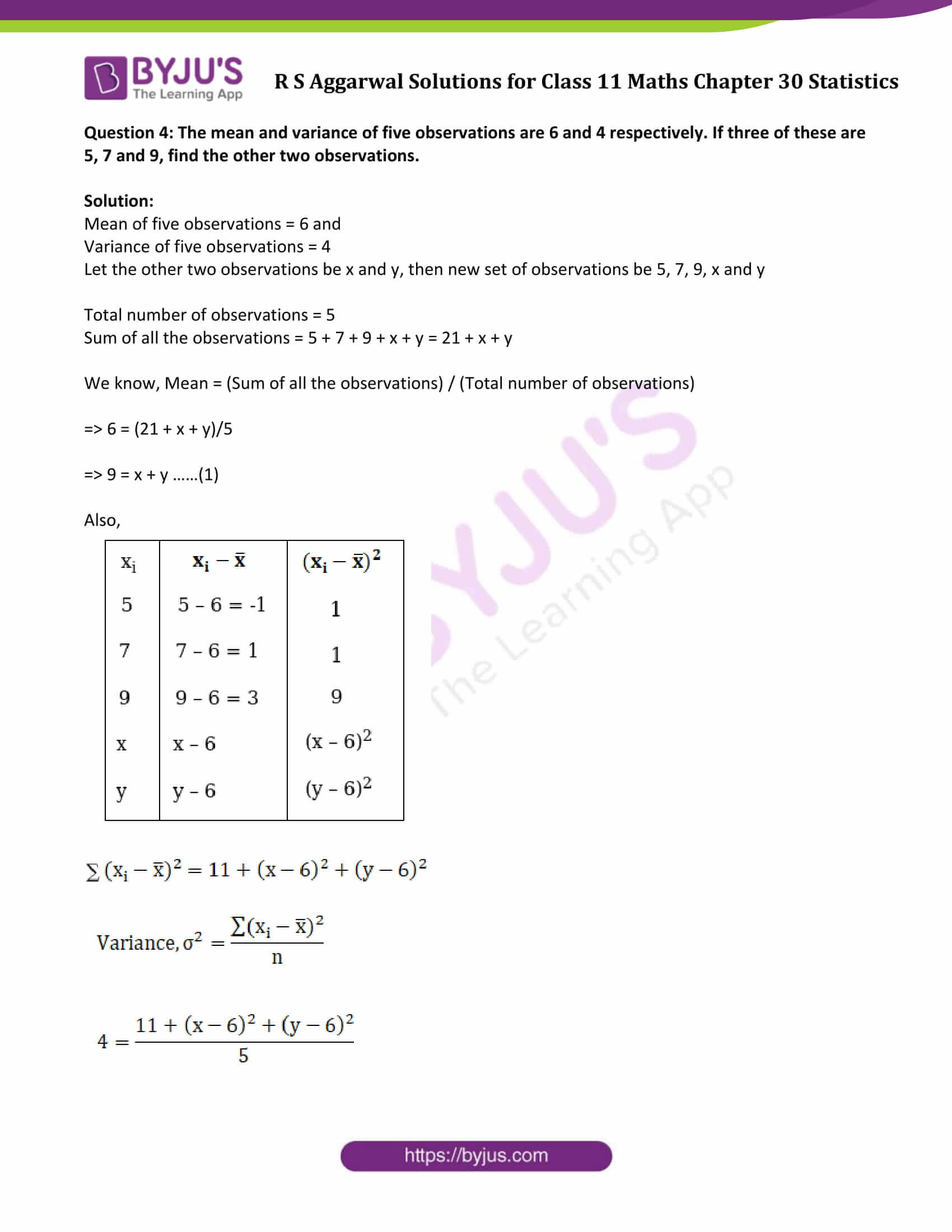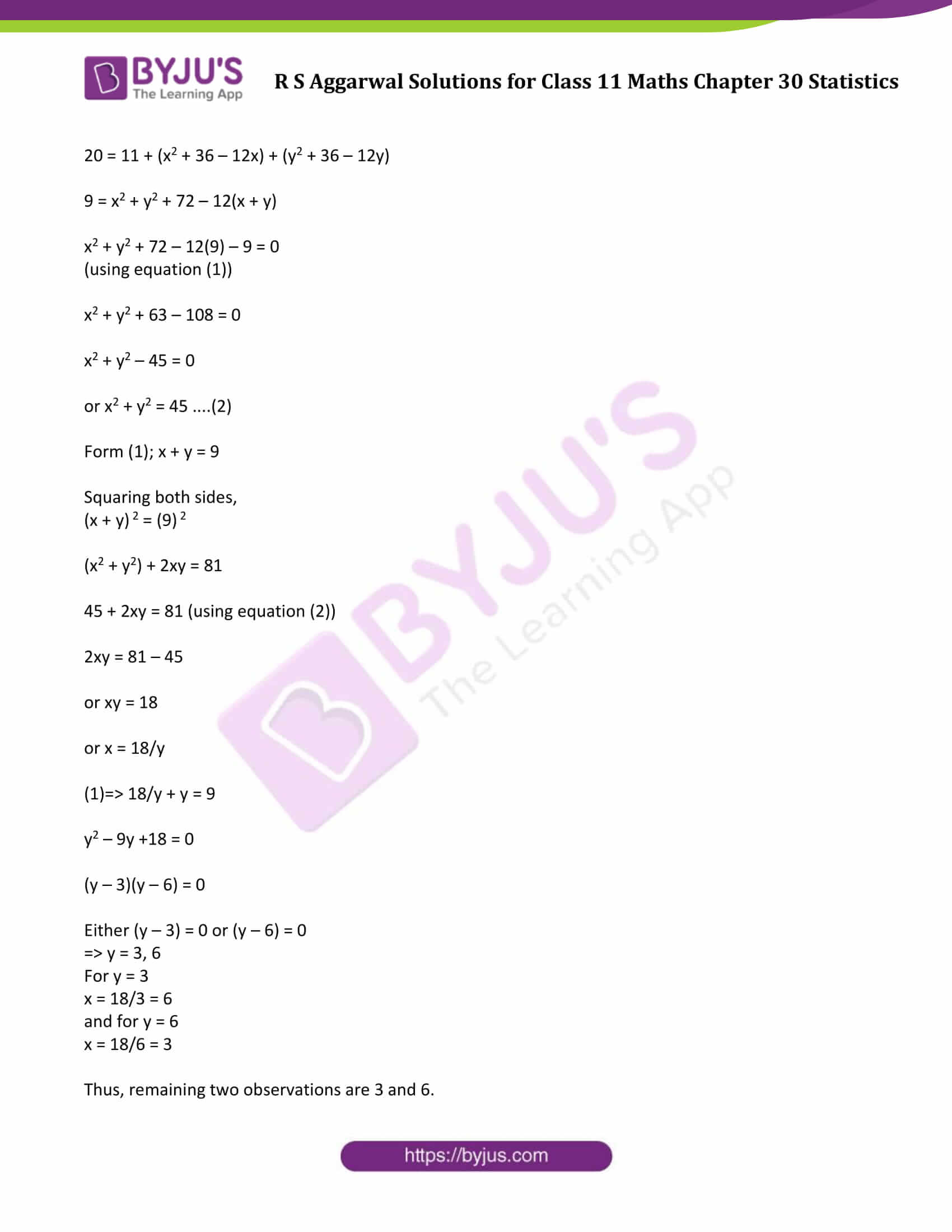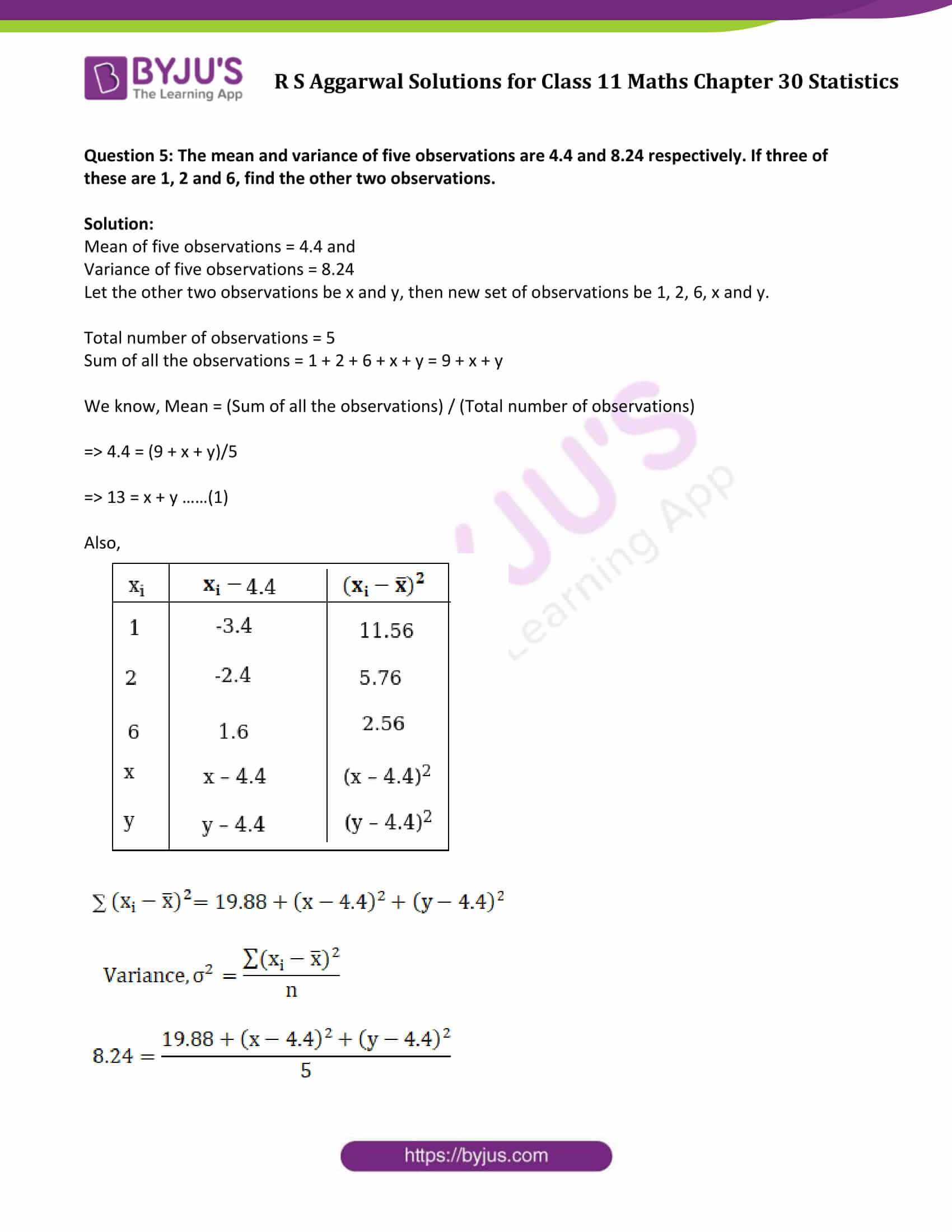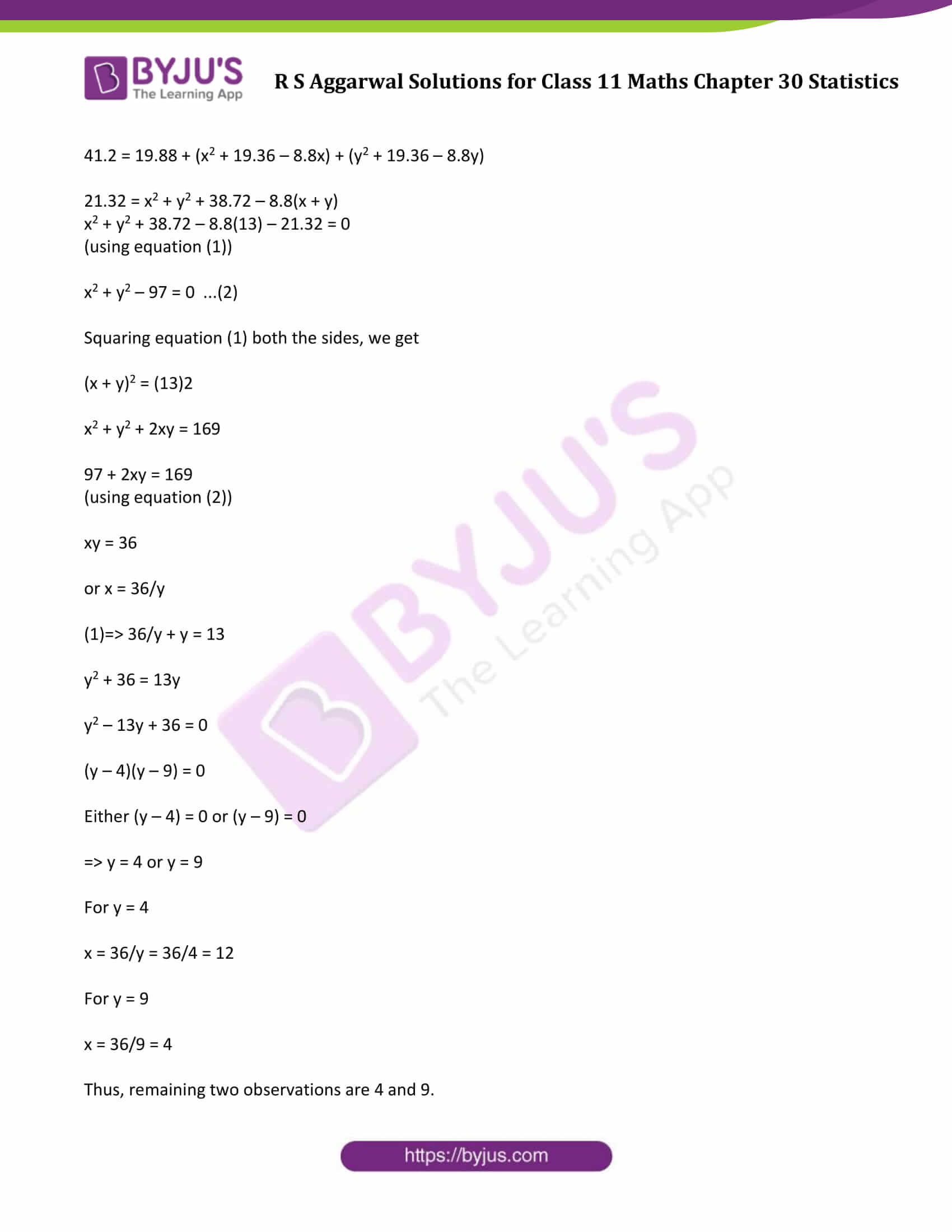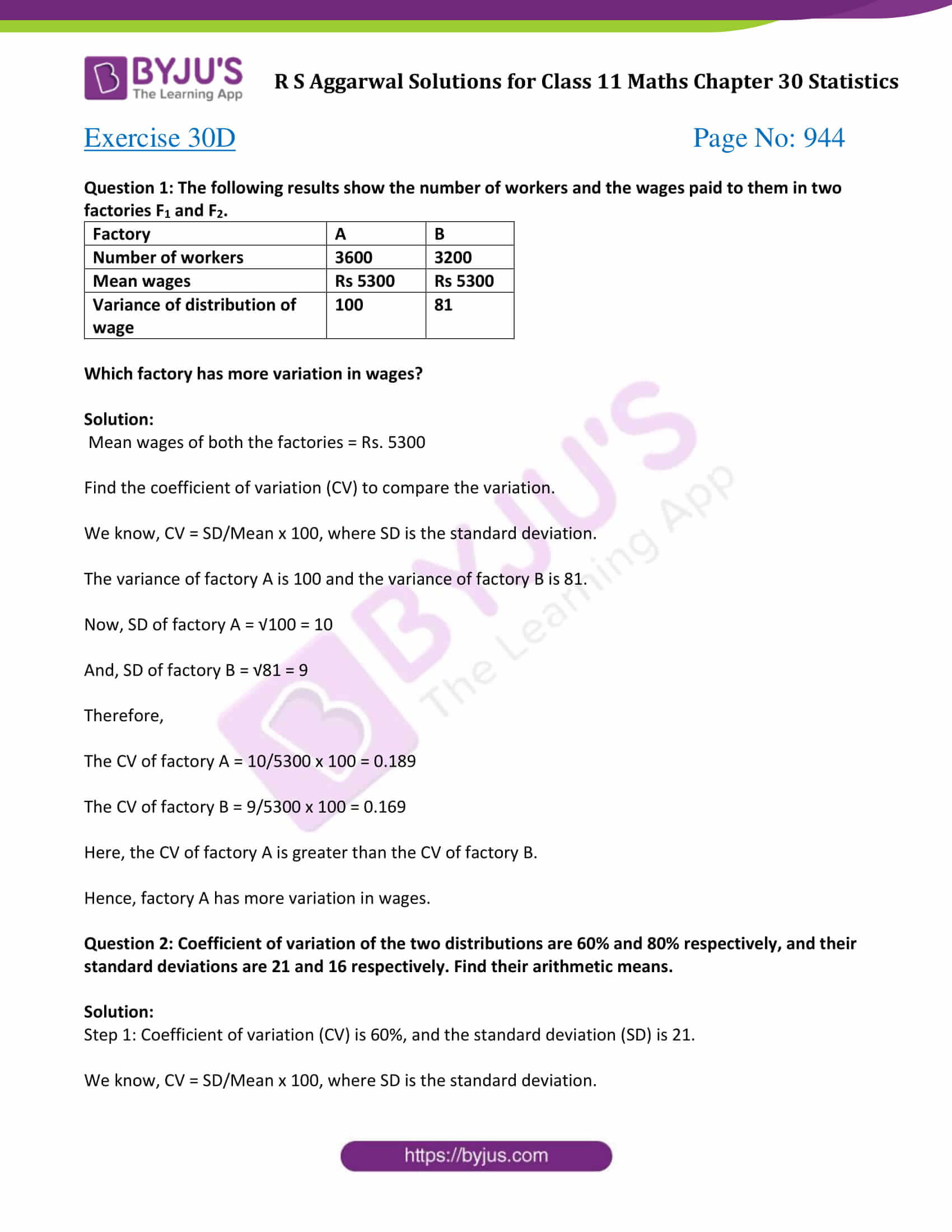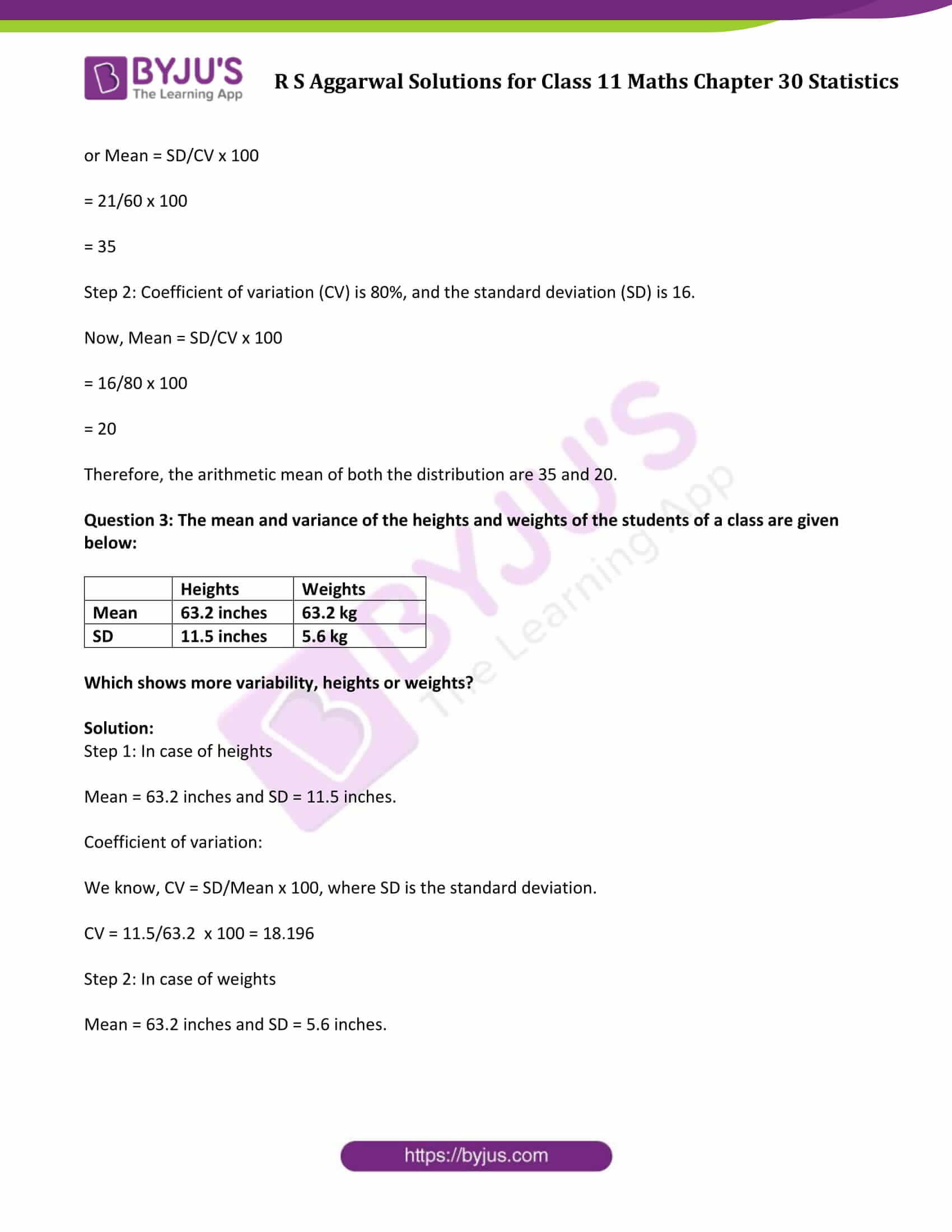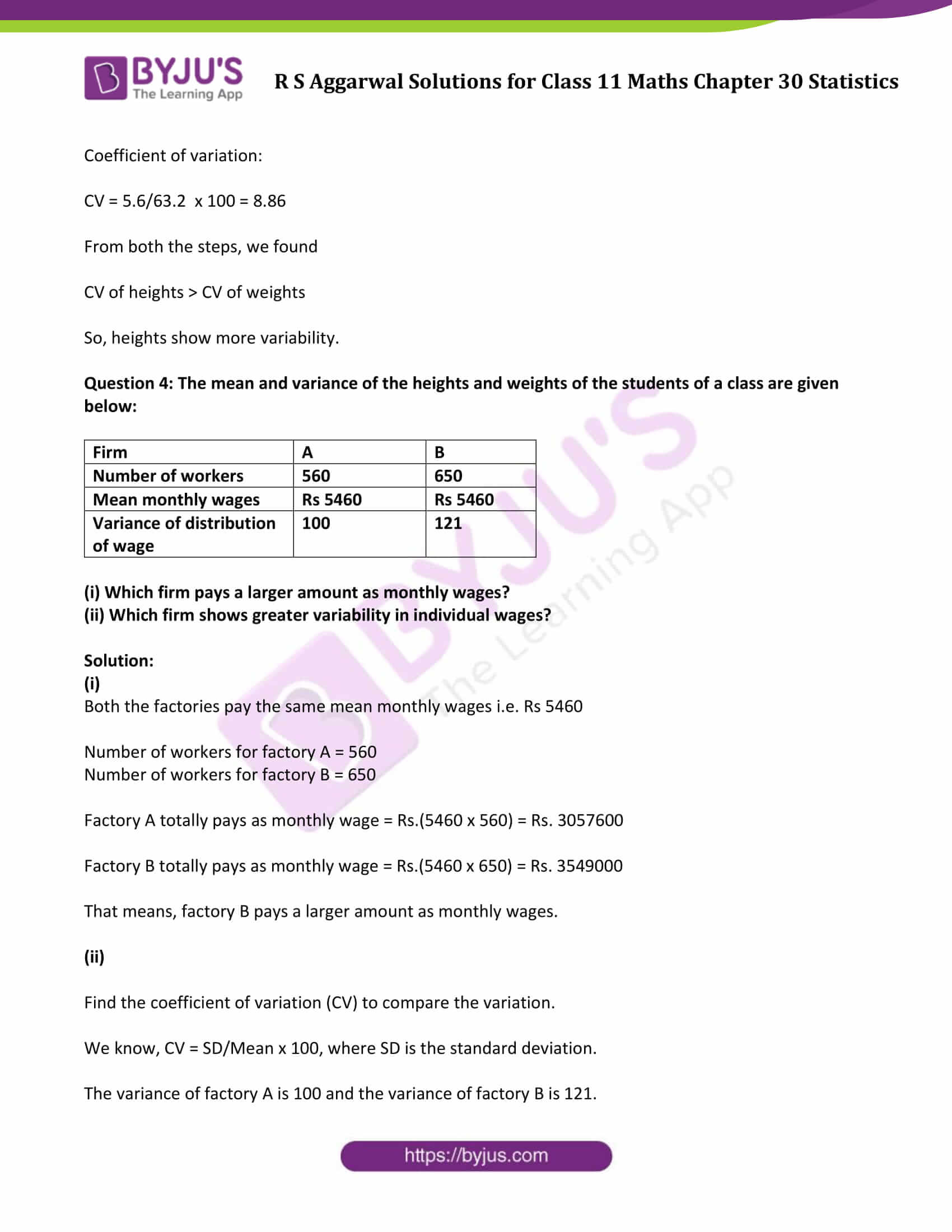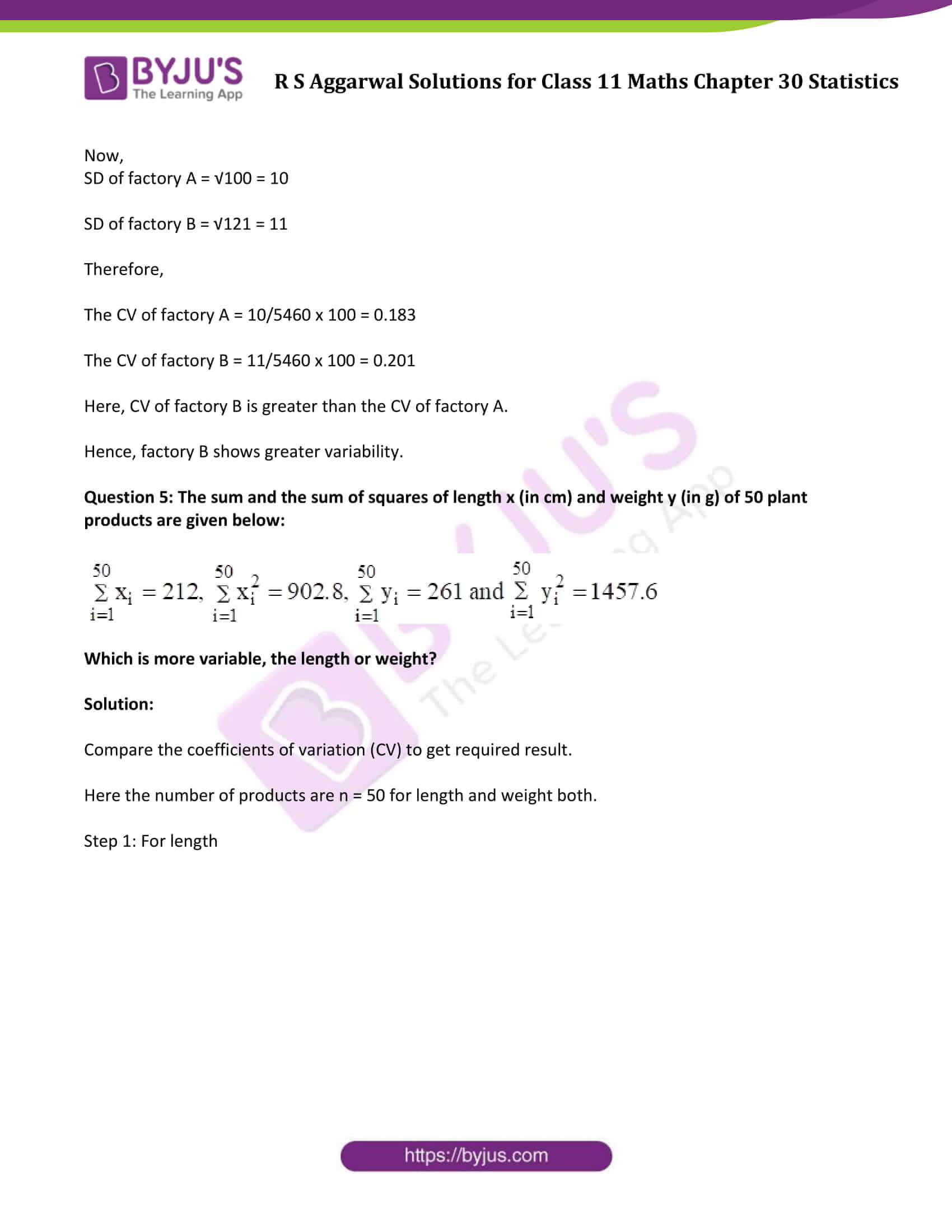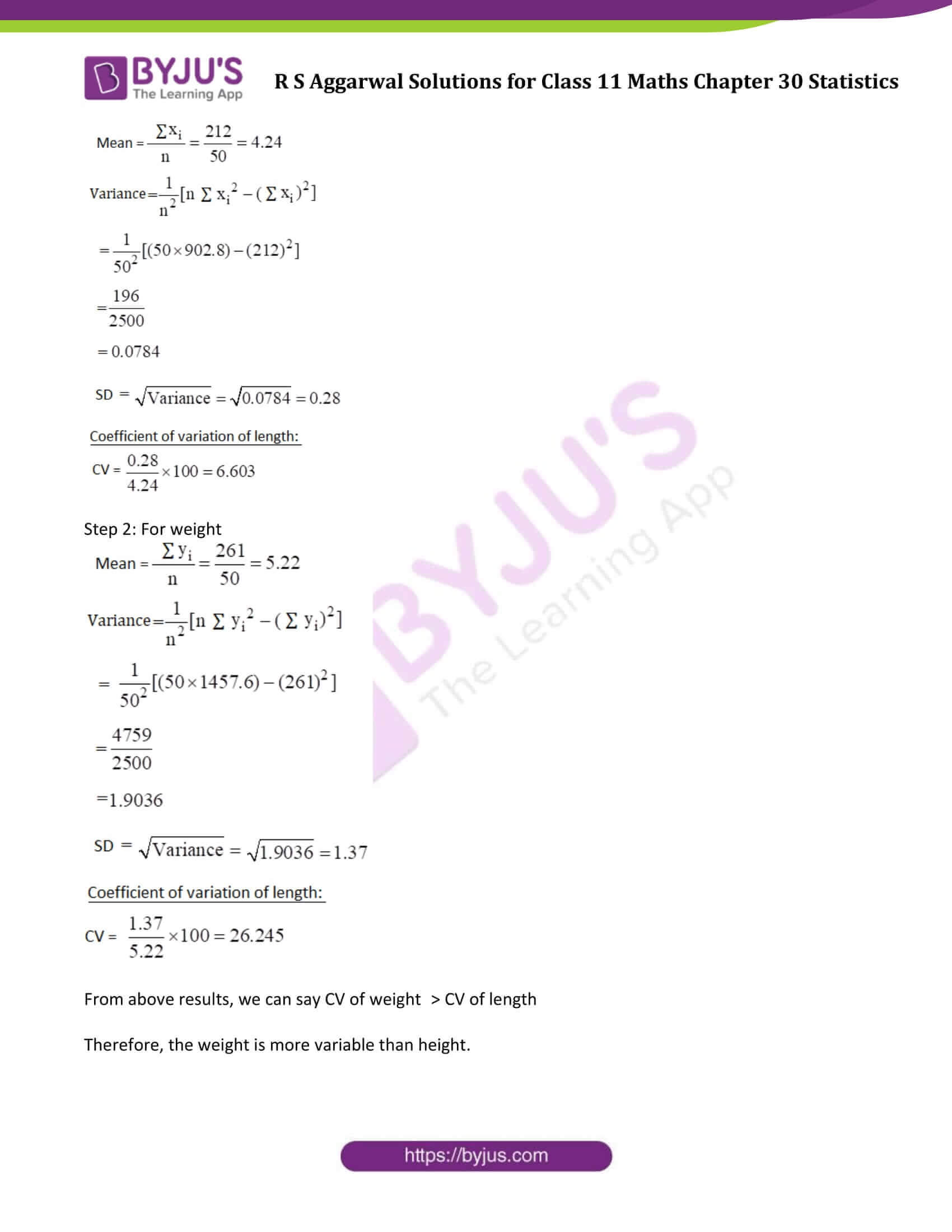### Access Answers to Maths R S Aggarwal Chapter 30 Statistics

Exercise 30A Page No: 926

Find the mean deviation about the mean for the following data: (Question 1 to Question 3)

Formula used: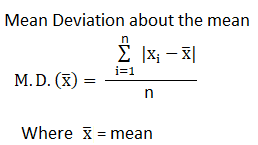Question 1: Find the mean deviation about the mean for 7, 8, 4, 13, 9, 5, 16, 18

Solution:

Step 1: Find the mean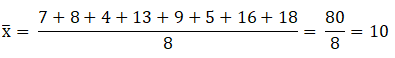Step 2: Mean deviation using formula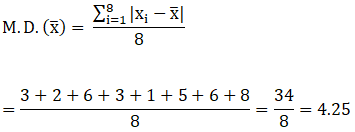Question 2: Find the mean deviation about the mean for 39, 72, 48, 41, 43, 55, 60, 45, 54, 43.

Solution:

Step 1: Find the mean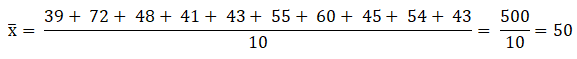Step 2: Mean deviation using formula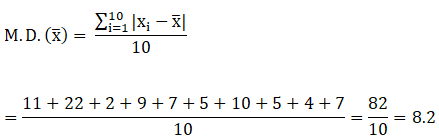Question 3: Find the mean deviation about the mean for 17, 20, 12, 13, 15, 16, 12, 18, 15, 19, 12, 11.

Solution:

Step 1: Find the mean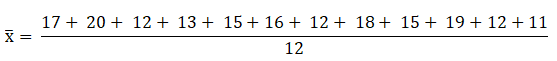= 180/12 = 15

Step 2: Mean deviation using formula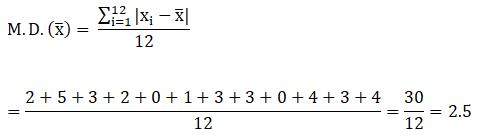Find the mean deviation about the median for the following data: (Question 4 to Question 7)

Formula used: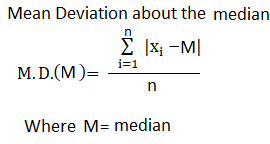Question 4: Find the mean deviation about the median for 12, 5, 14, 6, 11, 13, 17, 8, 10.

Solution:

Step 1: Find the median

Arranging the data into ascending order:

5, 6, 8, 10, 11, 12, 13, 14, 17

Total number of observations = 9, which is odd.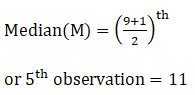Step 2: Mean deviation using formula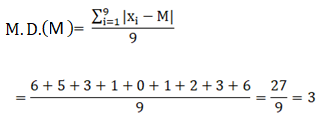Question 5: Find the mean deviation about the median for 4, 15, 9, 7, 19, 13, 6, 21, 8, 25, 11.

Solution:

Step 1: Find the median

Arranging the data into ascending order:

4, 6, 7, 8, 9, 11, 13, 15, 19, 21, 25

Total number of observations = 11, which is odd.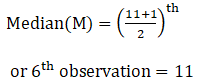Step 2: Mean deviation using formula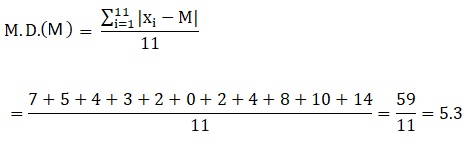Question 6: Find the mean deviation about the median for 34, 23, 46, 37, 40, 28, 32, 50, 35, 44.

Solution:

Step 1: Find the median

Arranging the data into ascending order:

23, 28, 32, 34, 35, 37, 40, 44, 46, 50

Total number of observations = 10, which is Even.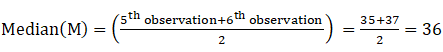Step 2: Mean deviation using formula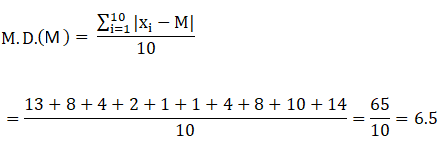Question 7: Find the mean deviation about the median for 70, 34, 42, 78, 65, 45, 54, 48, 67, 50, 56, 63.

Solution:

Step 1: Find the median

Arranging the data into ascending order:

34, 42, 45, 48, 50, 54, 56, 63, 65, 67, 70, 78

Total number of observations = 12, which is Even.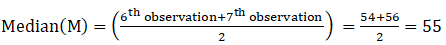Step 2: Mean deviation using formula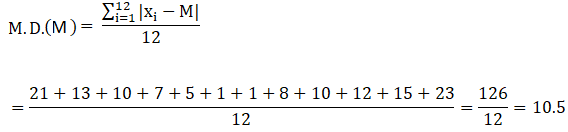Find the mean deviation about the mean for the following data: (Question 8 to Question 10)

Question 8: Find the mean deviation about the mean for below data:

 xi 6 12 18 24 30 36 fi 5 4 11 6 4 6

Solution: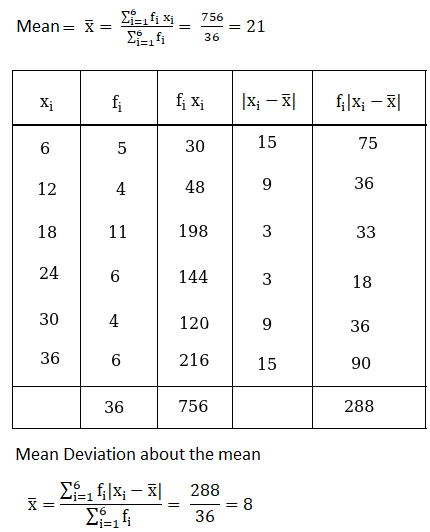Question 9: Find the mean deviation about the mean for below data:

 xi 2 5 6 8 10 12 fi 2 8 10 7 8 5

Solution:Question 10: Find the mean deviation about the mean for below data:

 xi 3 5 7 9 11 13 fi 6 8 15 25 8 4

Solution: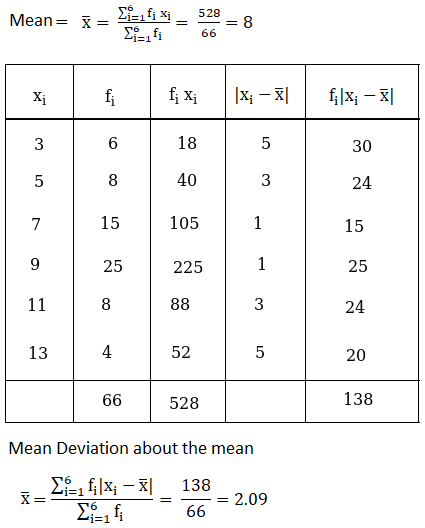Exercise 30B Page No: 935

Question 1: Find the mean, variance and standard deviation for the numbers 4, 6, 10, 12, 7, 8, 13, 12.

Solution:

Given data: 4, 6, 10, 12, 7, 8, 13, 12

Sum of observations = 4 + 6 + 10 + 12 + 7 + 8 + 13 + 12 = 72

Total number of observation = 8

Find Mean:

Mean = (Sum of observations) / (Total number of observation)

= 72/8 = 9

Mean = 9

Find Variance: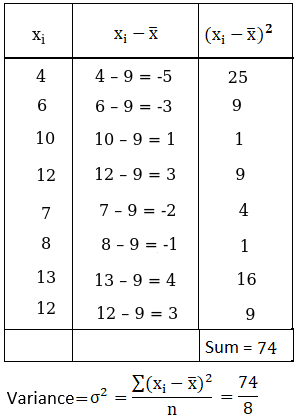Find Standard Deviation: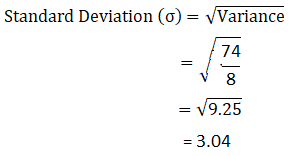Question 2: Find the mean, variance and standard deviation for first six odd natural numbers.

Solution:

Given data: First six odd natural numbers = 1, 3, 5, 7, 9, 11

Sum of observations = 1 + 3 + 5 + 7 + 9 + 11 = 36

Total number of observation = 6

Find Mean:

Mean = (Sum of observations) / (Total number of observation)

= 36/6 = 6

Mean = 6

Find Variance: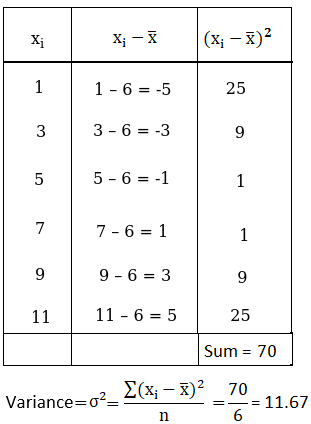Find Standard Deviation: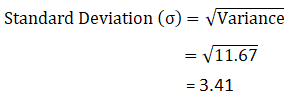Question 3: Using short cut method, find the mean, variance and standard deviation for the data :

 xi 4 8 11 17 20 24 32 fi 3 5 9 5 4 3 1

Solution: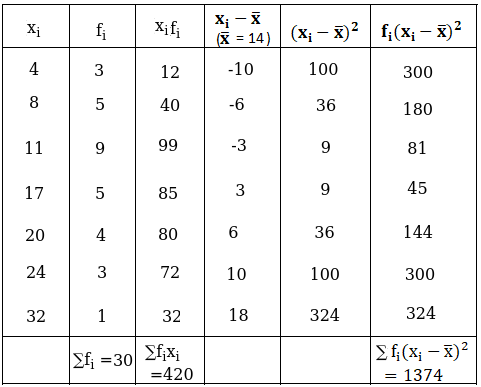Mean: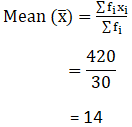Variance: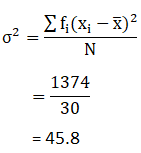Standard deviation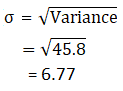Question 4: Using short cut method, find the mean, variance and standard deviation for the data :

 xi 6 10 14 18 24 28 30 fi 2 4 7 12 8 4 3

Solution: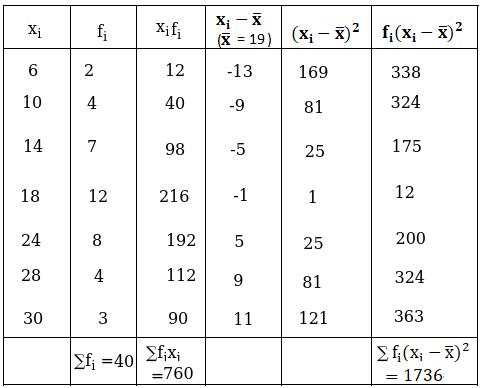Mean: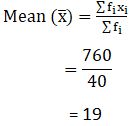Variance: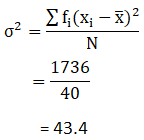Standard deviation: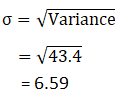Question 5: Using short cut method, find the mean, variance and standard deviation for the data :

 xi 10 15 18 20 25 fi 3 2 5 8 2

Solution: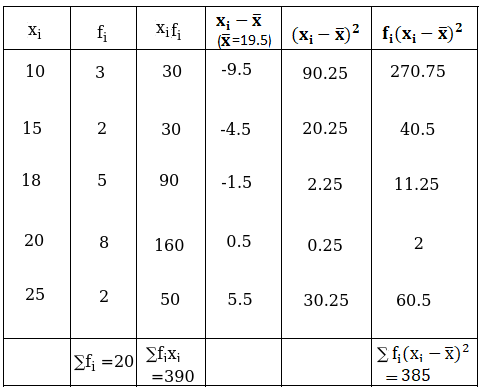Mean: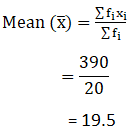Variance: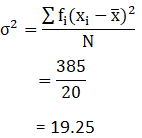Standard deviation: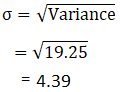Question 6: Using short cut method, find the mean, variance and standard deviation for the data :

 xi 92 93 97 98 102 104 109 fi 3 2 3 2 6 3 3

Solution: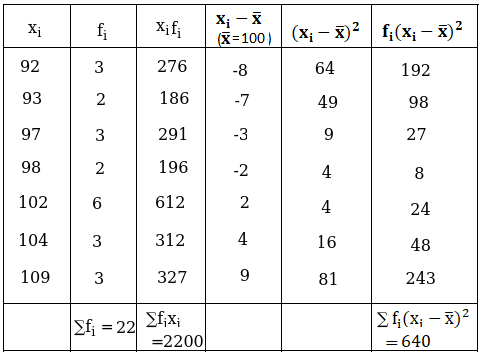Mean: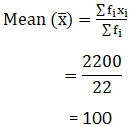Variance: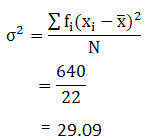Standard deviation: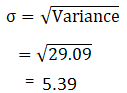Exercise 30C Page No: 941

Question 1: If the standard deviation of the numbers 2, 3, 2x, 11 is 3.5, calculate the possible values of x.

Solution:

Standard Deviation (σ) = 3.5

Sum of observations: 2 + 3 + 2x + 11 = 16 + 2x

Total number of observations = 4

Mean = (16+2x)/4 = (8+x)/2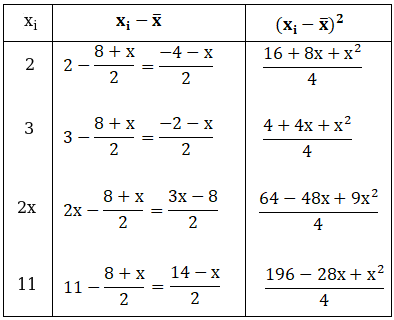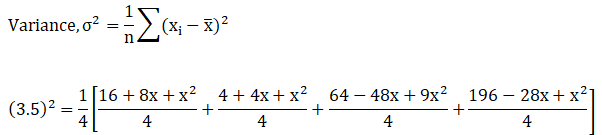12.25 × 16 = 280 – 64x + 12x2

196 = 280 – 64x + 12x2

12x2 – 64x + 84 = 0

or 3x2 – 16x + 21 = 0

or (3x – 7)(x – 3) = 0

=> Either 3x – 7 = 0 or x – 3 = 0

=>x = 7/3 or x = 3

Therefore, possible values of x are 3 and 7/3.

Question 2: The variance of 15 observations is 6. If each observation is increased by 8, find the variance of the resulting observations.

Solution:
Let x1, x2, x3, x4,….., x15 are any random 15 observations.

Variance = 6 and n = 15 (Given)

We know that,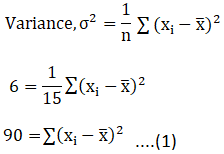If each observation is increased by 8, then let new observations be y1, y2, y3, y4,………, y15;

where yi = xi + 8 ….…(2)

Now, find the variance for new observations: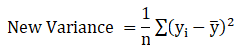Mean of new observations,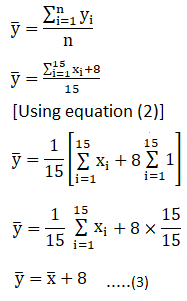Using equation (2) and (3) in equation (1), we get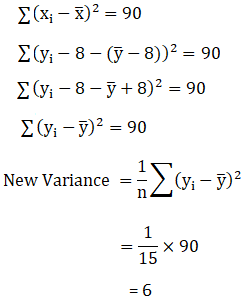Question 3: The variance of 20 observations is 5. If each observation is multiplied by 2. Find the variance of the resulting observations.

Solution:

Given: Variance = 5 and n = 20

Let x1, x2, x3, x4,….., x20 are any random 20 observations.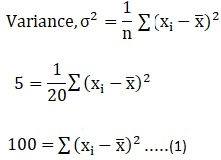If each observation is multiplied by 2, then let new observations be y1, y2, y3, y4,………, y20.

where yi = 2xi ……..(2)

Now, find the variance for new observations:Mean of new observations,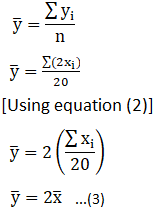Using equation (2) and (3) in equation (1), we get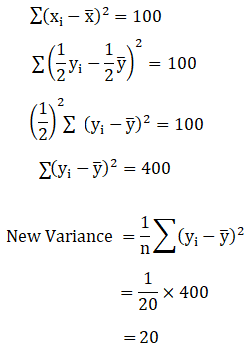Question 4: The mean and variance of five observations are 6 and 4 respectively. If three of these are 5, 7 and 9, find the other two observations.

Solution:

Mean of five observations = 6 and

Variance of five observations = 4

Let the other two observations be x and y, then new set of observations be 5, 7, 9, x and y

Total number of observations = 5

Sum of all the observations = 5 + 7 + 9 + x + y = 21 + x + y

We know, Mean = (Sum of all the observations) / (Total number of observations)

=> 6 = (21 + x + y)/5

=> 9 = x + y ……(1)

Also,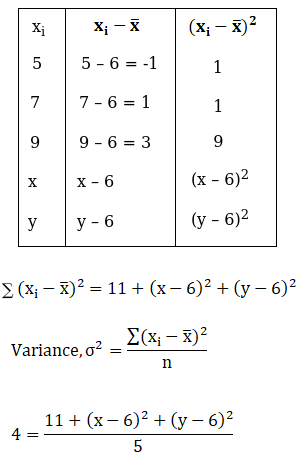20 = 11 + (x2 + 36 – 12x) + (y2 + 36 – 12y)

9 = x2 + y2 + 72 – 12(x + y)

x2 + y2 + 72 – 12(9) – 9 = 0

(using equation (1))

x2 + y2 + 63 – 108 = 0

x2 + y2 – 45 = 0

or x2 + y2 = 45 ….(2)

Form (1); x + y = 9

Squaring both sides,

(x + y) 2 = (9) 2

(x2 + y2) + 2xy = 81

45 + 2xy = 81 (using equation (2))

2xy = 81 – 45

or xy = 18

or x = 18/y

(1)=> 18/y + y = 9

y2 – 9y +18 = 0

(y – 3)(y – 6) = 0

Either (y – 3) = 0 or (y – 6) = 0

=> y = 3, 6

For y = 3

x = 18/3 = 6

and for y = 6

x = 18/6 = 3

Thus, remaining two observations are 3 and 6.

Question 5: The mean and variance of five observations are 4.4 and 8.24 respectively. If three of these are 1, 2 and 6, find the other two observations.

Solution:

Mean of five observations = 4.4 and

Variance of five observations = 8.24

Let the other two observations be x and y, then new set of observations be 1, 2, 6, x and y.

Total number of observations = 5

Sum of all the observations = 1 + 2 + 6 + x + y = 9 + x + y

We know, Mean = (Sum of all the observations) / (Total number of observations)

=> 4.4 = (9 + x + y)/5

=> 13 = x + y ……(1)

Also,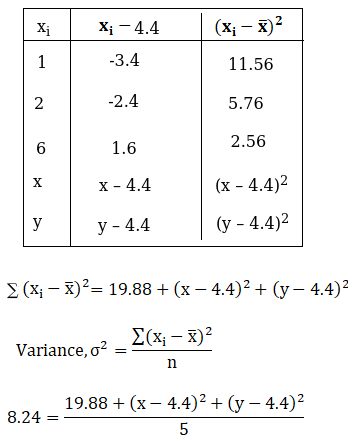41.2 = 19.88 + (x2 + 19.36 – 8.8x) + (y2 + 19.36 – 8.8y)

21.32 = x2 + y2 + 38.72 – 8.8(x + y)

x2 + y2 + 38.72 – 8.8(13) – 21.32 = 0

(using equation (1))

x2 + y2 – 97 = 0 …(2)

Squaring equation (1) both the sides, we get

(x + y)2 = (13)2

x2 + y2 + 2xy = 169

97 + 2xy = 169

(using equation (2))

xy = 36

or x = 36/y

(1)=> 36/y + y = 13

y2 + 36 = 13y

y2 – 13y + 36 = 0

(y – 4)(y – 9) = 0

Either (y – 4) = 0 or (y – 9) = 0

=> y = 4 or y = 9

For y = 4

x = 36/y = 36/4 = 12

For y = 9

x = 36/9 = 4

Thus, remaining two observations are 4 and 9.

Exercise 30D Page No: 944

Question 1: The following results show the number of workers and the wages paid to them in two factories F1 and F2.

 Factory A B Number of workers 3600 3200 Mean wages Rs 5300 Rs 5300 Variance of distribution of wage 100 81

Which factory has more variation in wages?

Solution:

Mean wages of both the factories = Rs. 5300

Find the coefficient of variation (CV) to compare the variation.

We know, CV = SD/Mean x 100, where SD is the standard deviation.

The variance of factory A is 100 and the variance of factory B is 81.

Now, SD of factory A = √100 = 10

And, SD of factory B = √81 = 9

Therefore,

The CV of factory A = 10/5300 x 100 = 0.189

The CV of factory B = 9/5300 x 100 = 0.169

Here, the CV of factory A is greater than the CV of factory B.

Hence, factory A has more variation in wages.

Question 2: Coefficient of variation of the two distributions are 60% and 80% respectively, and their standard deviations are 21 and 16 respectively. Find their arithmetic means.

Solution:

Step 1: Coefficient of variation (CV) is 60%, and the standard deviation (SD) is 21.

We know, CV = SD/Mean x 100, where SD is the standard deviation.

or Mean = SD/CV x 100

= 21/60 x 100

= 35

Step 2: Coefficient of variation (CV) is 80%, and the standard deviation (SD) is 16.

Now, Mean = SD/CV x 100

= 16/80 x 100

= 20

Therefore, the arithmetic mean of both the distribution are 35 and 20.

Question 3: The mean and variance of the heights and weights of the students of a class are given below:

 Heights Weights Mean 63.2 inches 63.2 kg SD 11.5 inches 5.6 kg

Which shows more variability, heights or weights?

Solution:

Step 1: In case of heights

Mean = 63.2 inches and SD = 11.5 inches.

Coefficient of variation:

We know, CV = SD/Mean x 100, where SD is the standard deviation.

CV = 11.5/63.2 x 100 = 18.196

Step 2: In case of weights

Mean = 63.2 inches and SD = 5.6 inches.

Coefficient of variation:

CV = 5.6/63.2 x 100 = 8.86

From both the steps, we found

CV of heights > CV of weights

Question 4: The mean and variance of the heights and weights of the students of a class are given below:

 Firm A B Number of workers 560 650 Mean monthly wages Rs 5460 Rs 5460 Variance of distribution of wage 100 121

(i) Which firm pays a larger amount as monthly wages?

(ii) Which firm shows greater variability in individual wages?

Solution:

(i)

Both the factories pay the same mean monthly wages i.e. Rs 5460

Number of workers for factory A = 560

Number of workers for factory B = 650

Factory A totally pays as monthly wage = Rs.(5460 x 560) = Rs. 3057600

Factory B totally pays as monthly wage = Rs.(5460 x 650) = Rs. 3549000

That means, factory B pays a larger amount as monthly wages.

(ii)

Find the coefficient of variation (CV) to compare the variation.

We know, CV = SD/Mean x 100, where SD is the standard deviation.

The variance of factory A is 100 and the variance of factory B is 121.

Now,

SD of factory A = √100 = 10

SD of factory B = √121 = 11

Therefore,

The CV of factory A = 10/5460 x 100 = 0.183

The CV of factory B = 11/5460 x 100 = 0.201

Here, CV of factory B is greater than the CV of factory A.

Hence, factory B shows greater variability.

Question 5: The sum and the sum of squares of length x (in cm) and weight y (in g) of 50 plant products are given below: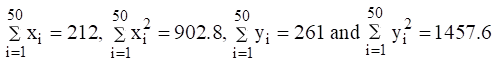Which is more variable, the length or weight?

Solution:

Compare the coefficients of variation (CV) to get required result.

Here the number of products are n = 50 for length and weight both.

Step 1: For length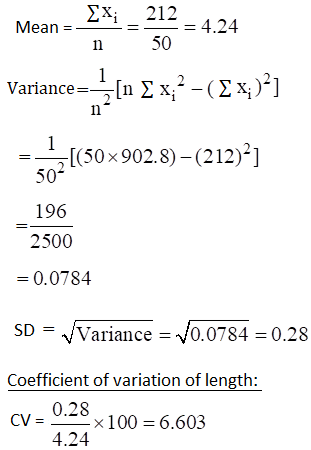Step 2: For weight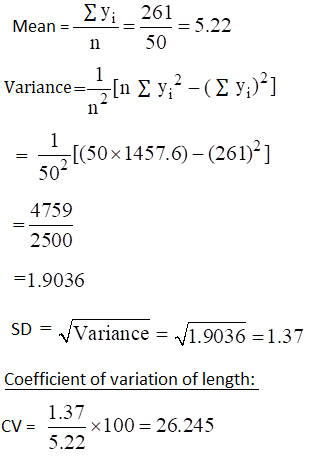From above results, we can say CV of weight > CV of length

Therefore, the weight is more variable than height.

## R S Aggarwal Solutions For Class 11 Maths Chapter 30 Exercises:

Get detailed solutions for all the questions listed under below exercises:

Exercise 30A Solutions

Exercise 30B Solutions

Exercise 30C Solutions

Exercise 30D Solutions